# JP5259797B2 - Learning type process abnormality diagnosis device and operator judgment estimation result collection device - Google Patents

Learning type process abnormality diagnosis device and operator judgment estimation result collection device Download PDF

## Info

Publication number
JP5259797B2
JP5259797B2 JP2011193219A JP2011193219A JP5259797B2 JP 5259797 B2 JP5259797 B2 JP 5259797B2 JP 2011193219 A JP2011193219 A JP 2011193219A JP 2011193219 A JP2011193219 A JP 2011193219A JP 5259797 B2 JP5259797 B2 JP 5259797B2
Authority
JP
Japan
Prior art keywords
unit
operator
abnormal state
process
history
Prior art date
Legal status (The legal status is an assumption and is not a legal conclusion. Google has not performed a legal analysis and makes no representation as to the accuracy of the status listed.)
Active
Application number
JP2011193219A
Other languages
Japanese (ja)
Other versions
JP2012009064A (en
Inventor

Original Assignee

Priority date (The priority date is an assumption and is not a legal conclusion. Google has not performed a legal analysis and makes no representation as to the accuracy of the date listed.)
Filing date
Publication date
Application filed by 株式会社東芝 filed Critical 株式会社東芝
Priority to JP2011193219A priority Critical patent/JP5259797B2/en
Publication of JP2012009064A publication Critical patent/JP2012009064A/en
Application granted granted Critical
Publication of JP5259797B2 publication Critical patent/JP5259797B2/en
Application status is Active legal-status Critical
Anticipated expiration legal-status Critical

## Images

•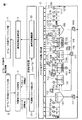•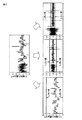•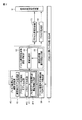•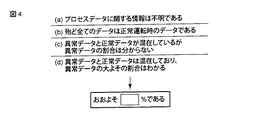•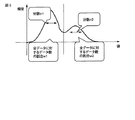•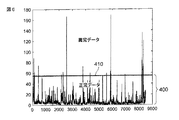•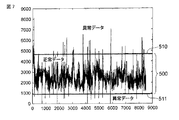••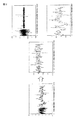••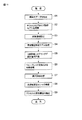•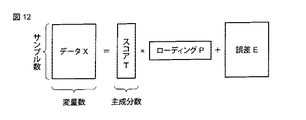•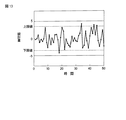•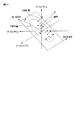•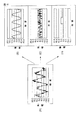•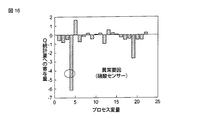•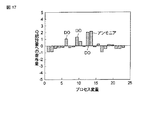•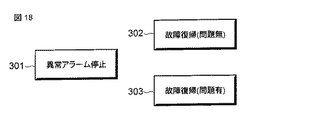•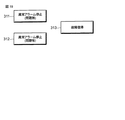••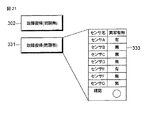•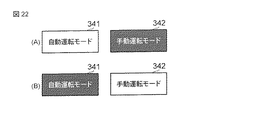•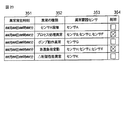•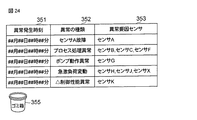•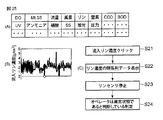••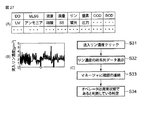•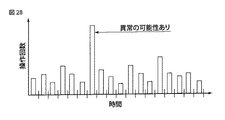•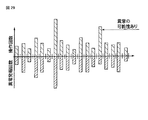•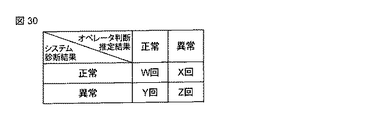•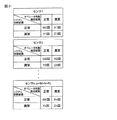•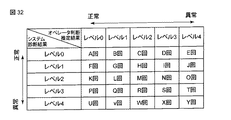•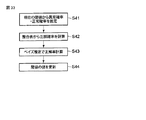•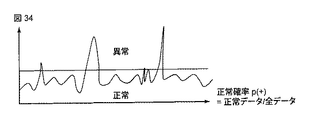•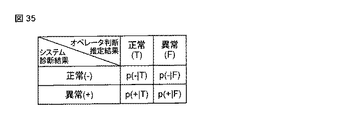•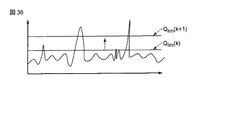•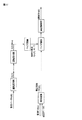•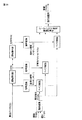•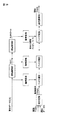•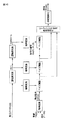•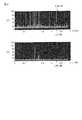•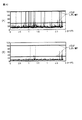## Description

The present invention particularly relates to a learning-type process abnormality diagnosis device for diagnosing a process system abnormality such as a water and sewage treatment process, a wastewater treatment process, a water purification process, or a chemical process, and an operator for estimating an operator's judgment in the process system The present invention relates to a judgment estimation result collection device.

In industrial processes such as water and sewage plants, nuclear power plants, chemical plants, and power plants, advanced process monitoring and process control aimed at reducing risks, saving energy, saving costs, saving people, etc. are required. It has become to. In particular, in process monitoring, it is necessary to predict various abnormal conditions, to quickly detect abnormal conditions, and to clarify the procedures for dealing with them. For example, in process operation management, it is appropriate for various abnormal situations such as abnormal sensor values, abnormal operation of devices such as actuators, sudden load fluctuations (sudden disturbances), abnormal process processing, abnormal control systems, etc. There is a need to deal with. In addition to daily process operation, predict failure of various equipment such as control infrastructure, power substations, pumps, blowers, etc. related to plant operation, and update equipment based on equipment status instead of time base There are many requirements for Condition Based Maintenance (CBM), and in many industrial fields such as water and sewage and nuclear power plant fields, it is expected that the time base maintenance, ie, Time Based Maintenance (TBM) will be switched to CBM in the future. Yes.

A technology that meets such a requirement is generally called “abnormality monitoring / diagnostic technology”. Abnormality monitoring / diagnosis techniques are widely used in various fields, and in a specific field, a technique called by a term specific to the specific field is used. For example, in the field of quality engineering that guarantees quality so that non-standard products (= abnormal products) are not produced in the production process, a method called Statistical Quality Control (SQC) is used. Although a method called statistical process control (SPC) is often used, this SPC is a kind of abnormality diagnosis technique. Further, as a diagnostic method well known in the field of quality engineering, there is an abnormality diagnostic technique called Mahalanobis-Taguchi method (MT method). For example, it is used in industrial fields such as management of manufacturing processes (overview management and quality change (corrosion etc.) management), medical fields (health checkups, etc.), management fields and the like. Also, in the field of chemical processes centered on petrochemical plants, the above-mentioned statistical process management SPC has been expanded when there are multiple sensor information, multivariate statistical process control (MSPC) and The method called is known (for example, refer nonpatent literature 1).

MSPC is sometimes called a chemometrics method, and is an abnormality diagnosis method developed in the field of chemical processes. As the most basic and frequently used method in MSPC, principal component analysis (PCA: Principal) There are methods called Component Analysis and Partial Least Square (PLS). In the field of mechanical systems and electrical systems, the dynamics of the target device is modeled using differential equations or difference equations, and based on this model, an estimation mechanism called an observer or Kalman filter is constructed to output the output from this estimation mechanism. A method of observing the difference between actual sensor outputs is often used. The method of making a diagnosis using not only the measured value of the sensor but also the calculated value of the model using the mathematical model in this way is sometimes called an analytical redundancy (AR) method. Also, in industrial fields related to mechanical / electrical systems, such as elevators and traffic systems, the signals measured by sensors (Fast Fourier Transform (FFT)) and wavelet transforms (WT: Wavelet). In many cases, abnormality diagnosis is performed by performing signal processing such as Transform, DWT: Discrete Wavelet Transform, and CWT: Continuous Wavelet Transform) and various digital filters.

As described above, abnormality diagnosis technology is widely used in various fields, and there is a specific method called by a unique term in a specific field. However, even if any abnormality diagnosis technology is applied, there is always a problem. It becomes a problem of the performance of abnormality diagnosis. Normally, the performance of abnormality diagnosis is the number (or rate) of false alarms (which are actually abnormal but cannot be detected) and false alarms (which are actually normal but diagnosed as abnormal). Often evaluated, reducing the number of miss alarms and false alarms as much as possible will improve the performance of the abnormality diagnosis technology. However, there is often a trade-off between the reduction in miss alarms and the reduction in false alarms. Because, regardless of what abnormality diagnosis technology is applied, it is normal to set a standard parameter (usually threshold = threshold) that will normally determine whether an abnormality is normal.・ This is because abnormalities are often judged.

If this parameter (threshold) is set to reduce the number of false alarms, the possibility of false alarms increases, and conversely, if it is set to reduce the number of false alarms, the possibility of false alarms Becomes higher. Therefore, optimizing the setting level of such parameters (threshold values) is a very important issue in the abnormality diagnosis system. In order to set an appropriate threshold value, engineers who build the target abnormality diagnosis system often make adjustments through trial and error, but this method depends on the individual engineer's ability to make adjustments. There is a problem that a large number of man-hours are required when the number of threshold values to be set increases.

On the other hand, various methods for automatically setting an appropriate value of the threshold based on some calculation have been proposed. For example, in the field of quality control, assuming the normality of data, it is often managed with 3σ based on the estimated value of standard deviation σ, which is called a control limit value and is a kind of threshold value. . In MSPC, data called Q statistics and data called T 2 statistics are used as data for detecting anomalies. As for the Q statistic that approximately follows the χ 2 distribution, it is generally performed by approximating the Q statistic (which is converted from) with a normal distribution and using the confidence limit value as a threshold value.

For the T 2 statistic, a threshold value is generally determined by a test using an F distribution. In the field of failure diagnosis of equipment and the like, determination is also made by setting a threshold value indirectly based on a test such as a likelihood ratio test.

While these methods have the advantage that threshold values can be uniformly set based on objective criteria, such objective setting methods are often viewed from the user (plant operator) of an abnormality diagnosis system. The threshold that seems inappropriate may be set, and any (automatic) setting method cannot be used to satisfy the user of the abnormality diagnosis system (plant operator in the case of plant operation). There are many cases. This is because the appropriateness of the threshold setting ultimately depends on the judgment of the user (plant operator) who uses the abnormality diagnosis system in many cases.

The most important reason why the “appropriate threshold setting value” is not obtained by objective calculation and depends on the user's judgment is that, in order to correctly define miss alarms and false alarms, ”And“ really abnormal state ”must be defined correctly, but in a real process,“ really normal state ”and“ really abnormal state ”may not always be defined correctly. In other words, there is a gray (fuzzy) state between the “really normal state” and the “really abnormal state” that is “normal or abnormal”, and this gray state is In many cases, it is impossible to determine whether or not it is determined as “normal” based on objective facts. Do you think "I want you to report as an anomaly even in a gray state", or "Do you think that a gray state is reported as an anomaly one by one, the anomaly reports become too much and process management becomes difficult?" It is a problem that depends essentially on subjective judgment. The threshold value usually needs to be set within a range considered to be this gray state. This is because it should not be judged as abnormal when it is clearly in a normal state, but the value of the abnormality diagnosis system is lost even if a diagnosis of abnormality in the abnormal state is clearly made. (Because it diagnoses that the state has deviated from the normal state before a failure (abnormality) occurs.) For a gray state, one simple example in which the diagnostic threshold varies depending on the subjectivity is shown.

FIG. 42 is time-series data of the valve opening degree of a blower (air supply device) in a certain sewage treatment plant. 42A and 42B show different series of valve opening degrees. In FIG. 42, the valve opening varies between 0% and 100%. In the series shown in FIG. 42A, the valve opening degree may increase relatively frequently. In the series shown in FIG. 42 (B), the valve opening is rarely increased. Consider that abnormality determination is performed for such data by simply setting a threshold value. In this case, in principle, there are the following four positions. Although it is possible in principle to include only abnormal data without including normal data, this position is excluded because it is impossible in reality.

(1) FIG. 42A and FIG. 42B do not include abnormal data. Therefore, the threshold value is set at a physically possible point.

(2) Both FIG. 42A and FIG. 42B include abnormal data. Therefore, the threshold value is set to an appropriate location of 0% to 100%.

(3) FIG. 42A does not include abnormal data, but FIG. 42B includes abnormal data. Therefore, in FIG. 42A, the threshold value is set to a point of 100%, but the threshold value of FIG. 42B is set to an appropriate location of 0% to 100%.

(4) FIG. 42A includes abnormal data, but FIG. 42B does not include abnormal data. Therefore, in FIG. 42A, the threshold value is set at an appropriate location of 0% to 100%, but the threshold value in the following diagram is set at a point of 100%.

Although these four positions can be taken, among them, it seems that few people take the position (4), but it is possible to take the other three positions.

The person who takes the position of (1) says, “Because the valve opening can be operated between 0% and 100% in the first place, there is no problem in moving within that range. All of the data are normal data. "

The person who takes the position of (2) says, “The valve opening can operate between 0% and 100%, but the data shows that the valve opening does not exceed 40% in FIG. 42 (B), it is unlikely to exceed 20%, so a value in the vicinity of this should be set as a threshold value, and if there is an opening larger than that, some (operation) abnormal operation will not occur. It should be regarded as having occurred. "

The person who takes the position of (3) says, “In FIG. 42 (A), the valve opening often increases to nearly 100%, but in FIG. 42 (B), the valve opening rarely increases. In the above figure, 100% should be set as the threshold value, but in FIG. 42 (B), it should be set to a lower value. Each of these positions has a certain level of persuasiveness, and it cannot be generally said which position is correct. Therefore, from the standpoint of realizing an abnormality diagnosis system, it is important to realize a mechanism that reflects such subjectivity. If this mechanism can be realized, a system that meets the preferences of users with various subjects will be constructed. be able to.

Manabu Kano, "Multivariate Statistical Process Management", June 2005 2nd Edition, Kyoto University Graduate School of Engineering, Department of Chemical Engineering, Process System Engineering Laboratory, [March 2, 2007 search], Internet <URL: http : //tech.chase-dream.com/spc/report-MSPC.pdf>

In order to accurately detect an abnormality in process abnormality diagnosis, it is not sufficient to simply increase the sensitivity to abnormality detection, and it is important to appropriately adjust the trade-off between a miss alarm and a false alarm. Although it is ideal to reduce both miss alarms and false alarms as much as possible, there is actually a duplication of abnormal and normal events in the boundary between normal and abnormal. Abnormality and normality are often not completely discernable. For this reason, it has been confirmed that the false alarm usually increases when the false alarm is reduced, and the false alarm increases when the false alarm is reduced.

Accordingly, an object of the present invention is to provide a learning type process abnormality diagnosis apparatus that achieves an appropriate abnormality diagnosis performance for actual process monitoring along with accurate abnormality detection by optimizing the trade-off between a miss alarm and a false alarm Is to provide.

A learning type process abnormality diagnosis apparatus according to an example of the present invention includes a diagnosis unit that diagnoses whether the target process is in a normal state or an abnormal state based on one or more adjustable parameters of the target process. A diagnosis result presentation unit for presenting a diagnosis result of the diagnosis unit to an operator, and whether the operator determines that the target process is in a normal state or an abnormal state, or the operator is input The operator judgment feedback unit that estimates the determination and outputs the input result or the estimation result, a history holding unit that holds a history of comparison information between the diagnosis result of the diagnosis unit and the output result of the operator determination feedback unit, , Adjustable parameter learning for learning a correction value of the adjustable parameter based on the history stored in the history holding unit And an adjustable parameter setting unit for setting the value of the adjustable parameter in the diagnostic unit based on the adjustable parameter learned by the adjustable parameter learning unit, and from the target process in accordance with an operation of the operator A process data history display unit for displaying a history of measured process data; and an operation unit for the operator to operate a device included in the target process. The operator determination feedback unit includes each process data and each The process data history display unit has information on correlation between devices, and the operator determination feedback unit is operated after the process data history display unit is operated in a state in which the diagnosis unit diagnoses that it is in a normal state. When a device having a high correlation with the process data displayed on the The operator is characterized in that to assume that it is determined that the abnormal state.

According to the present apparatus, it is possible to realize abnormality diagnosis performance suitable for practical process monitoring as well as accurate abnormality detection.

The block diagram which shows schematic structure of the learning type | mold abnormality diagnosis apparatus concerning one Embodiment of this invention. The figure which showed the parameter used for the concept of a sensor abnormality diagnosis method using a discrete wavelet transform, and abnormality diagnosis. The block diagram which shows the structure of the abnormality diagnosis function part using discrete wavelet transform. The figure for demonstrating the input method of the prior information for normalization regarding the abnormality diagnosis function part shown in FIG. The figure for demonstrating the concept of the threshold value determination method concerning the abnormality diagnosis function part shown in FIG. The figure which shows the concept for setting the threshold value which classifies the normal data and abnormality data which concern on the abnormality diagnosis function part shown in FIG. The figure which shows the concept for setting the threshold value which classifies the normal data and abnormality data which concern on the abnormality diagnosis function part shown in FIG. The figure which shows the specific example which decomposed | disassembled the Q statistic regarding the abnormality diagnosis function part shown in FIG. 3 into the data for every some frequency band by discrete wavelet transform. The figure for demonstrating the effect of the abnormality detection rate concerning the abnormality diagnosis function part shown in FIG. The block diagram which shows the structure of the abnormality diagnosis function part concerning one Embodiment of this invention. 11 is a flowchart for explaining a processing procedure of the abnormality diagnosis function unit shown in FIG. 10. The figure which shows the concept of the data decomposition | disassembly by PCA regarding 1st Embodiment regarding the abnormality diagnosis function part shown in FIG. The figure which shows the statistic data regarding the abnormality diagnosis function part shown in FIG. 10 as time series data with a control limit value. The figure for demonstrating the principle of the abnormality diagnosis using PCA regarding the abnormality diagnosis function part shown in FIG. The figure for demonstrating the decomposition | disassembly concept of the time series data by the wavelet transformation regarding the abnormality diagnosis function part shown in FIG. The figure which shows the specific example of the process abnormality factor sensor which can identify easily regarding the abnormality diagnostic function part shown in FIG. The figure which shows the specific example of the process abnormality factor sensor which is hard to identify regarding the abnormality diagnostic function part shown in FIG. The figure which shows the button for carrying out failure recovery, after stopping an abnormal alarm at the time of the abnormality alerting concerning one Embodiment of this invention. The figure which showed another button example for carrying out a failure recovery after stopping an abnormal alarm at the time of abnormality notification concerning one Embodiment of this invention. The figure which showed another example of a button which performs failure recovery concerning one Embodiment of this invention. The figure which showed another example of a button which performs failure recovery concerning one Embodiment of this invention. The figure which shows the state of the operation mode displayed on the monitoring screen concerning one Embodiment of this invention. The figure which showed the example which can display the log | history information of abnormal alerting alarm, and can delete arbitrary abnormal alerting alarms concerning one Embodiment of this invention. The figure which showed another example which can display the log | history information of an abnormal alarm alarm, and can delete arbitrary abnormal alarms concerning one Embodiment of this invention. The figure which showed the example of the action order which performs operation with respect to the apparatus relevant to a process, after confirming the time series data of the process data concerning one Embodiment of this invention. The figure which showed the example of the table | surface which matches the sensor which measures a certain process data, and the process apparatus relevant to the sensor concerning one Embodiment of this invention. The figure which showed the example of the action order which contacts by a communication means, after confirming the time series data of process data concerning one Embodiment of this invention. The figure explaining the concept which estimates the occurrence of abnormality based on the example which displayed the frequency | count of operation with respect to the operation amount for every predetermined | prescribed period. The figure explaining the concept which estimates the occurrence of an abnormality based on the example which displayed the balance of the frequency | count of operation for every predetermined period, and the frequency | count of abnormality report. The figure which showed the example of the table | surface (table) which put together the judgment result of the operator, and the diagnostic result of the abnormality diagnosis part. The figure which showed the example of the table | surface which put together the comparison result of the judgment result of an operator, and the diagnostic result of an abnormality diagnosis part for every sensor. The figure which showed the example of the table | surface which put together the judgment result of an operator and the diagnostic result of an abnormality diagnostic part for every sensor in case there exist multiple abnormality levels. The flowchart which shows the learning (adjustment) procedure of an adjustable parameter concerning one Embodiment of this invention. The figure for demonstrating the log | history of process data and normal probability concerning one Embodiment of this invention. The figure which shows the table | surface which shows four conditional probabilities concerning one Embodiment of this invention. The figure which shows the example of the update of a threshold value concerning one Embodiment of this invention. The figure shown for demonstrating an example of the learning procedure of an adjustable parameter concerning one Embodiment of this invention. The figure shown for demonstrating an example of the learning procedure of an adjustable parameter concerning one Embodiment of this invention. The figure shown for demonstrating an example of the learning procedure of an adjustable parameter concerning one Embodiment of this invention. The figure shown for demonstrating an example of the learning procedure of an adjustable parameter concerning one Embodiment of this invention. The figure explaining that the setting problem of the value of a scaling parameter can be handled similarly to the setting problem of a threshold value. The figure which shows that it is difficult to set the threshold value which judges abnormality and normality based on objective criteria.

Embodiments of the present invention will be described below with reference to the drawings.

FIG. 1 is a block diagram showing a schematic configuration of a learning type abnormality diagnosis apparatus according to an embodiment of the present invention.

The process abnormality diagnosis apparatus according to the present embodiment is used in a process monitoring system that monitors process 1 such as a sewage treatment process for the purpose of removing nitrogen and phosphorus, for example. Note that the process abnormality diagnosis apparatus of the present embodiment is not limited to the sewage treatment process as the process 1 to be monitored, but is applicable to other processes such as a chemical process, a waterworks plant, a nuclear power plant, and a power plant. it can.

[Sewage treatment process]
The sewage treatment process 1 includes a first sedimentation tank 101, a first anaerobic tank 102, a second aerobic tank 103, a third anaerobic tank 104, a fourth aerobic tank 105, and a final sedimentation tank 106. In addition, the sewage treatment process 1 includes an initial sedimentation pond excess sludge extraction pump 111 including an extraction flow sensor, an acetic acid input pump 112 that supplies acetic acid-based organic matter including an input amount sensor, a step inflow pump 113 that includes a step inflow sensor, and a supply A blower 114 for supplying oxygen to a second aerobic tank including an air flow sensor, a carbon source input pump 115 for supplying a carbon source (organic matter) including an input sensor, a circulation pump 116 including a circulation flow sensor, and a return flow sensor A return sludge pump 117 including a blower 118 for supplying oxygen to a fourth aerobic tank including a supply air flow rate sensor, a flocculant input pump 119 including an input amount sensor, and a final sedimentation tank surplus sludge extraction pump including an extraction flow rate sensor Each of 1110 has an actuator and its operation amount sensor group.

Further, the sewage treatment process 1 includes a sewage inflow sensor 121 that measures the inflow sewage amount, an inflow TN sensor 122 that measures the total nitrogen amount contained in the inflow sewage, and an inflow TP sensor that measures the total phosphorus amount contained in the inflow sewage. 123, an inflow UV (Ultra Violet) sensor or inflow COD (chemical oxygen demand) sensor 124 for measuring the amount of organic substances contained in the inflow sewage, a phosphate sensor 125 for measuring the phosphoric acid concentration in the first anaerobic tank 102, and a second preference An ammonia sensor 126 that measures the ammonia concentration in the air tank 103, a DO (dissolved oxygen) sensor 127 that measures the dissolved oxygen concentration in the second aerobic tank 103, and a nitric acid sensor 128 that measures the nitric acid concentration in the third oxygen-free tank 104. Have

The sewage treatment process 1 includes MLSS (mixed) that measures the amount of activated sludge in at least one of the first anaerobic tank 102, the second aerobic tank 103, the third anaerobic tank 104, and the fourth aerobic tank 105. liquor suspended solid) sensor 129, phosphoric acid sensor 1210 for measuring the phosphoric acid concentration in the fourth aerobic tank 105, DO (dissolved oxygen) sensor 1211 for measuring the dissolved oxygen concentration in the fourth aerobic tank 105, fourth aerobic The ammonia sensor 1212 for measuring the ammonia concentration in the tank 105, the MLSS sensor 1213 for measuring the MLSS concentration of the sludge drawn from the final sedimentation basin 106, and the suspended solid concentration of the effluent discharged from the final sedimentation basin 106 SS sensor 1214 for measuring, sewage discharge sensor 1215 for measuring the amount of discharged sewage, discharge TN sensor for measuring the total amount of nitrogen contained in the discharged sewage 216 have respective discharge UV sensor or discharged COD sensor 1218 measures the amount of organic matter contained in effluent TP sensor 1217, and sewage effluent to measure the total amount of phosphorus contained in the sewage effluent as process sensor.

Here, the various actuators 111 to 119 and 1110 described above operate at a predetermined cycle. Further, the operation amount sensor group of the various actuators 111 to 119 and 1110 and the various process sensors 121 to 129 and 1218 measure at a predetermined cycle.

[Learning type abnormality diagnosis device]
As shown in FIG. 1, the learning-type abnormality diagnosis apparatus includes a process data collection / storage unit 2 that collects data, an abnormality diagnosis unit 3, an abnormality diagnosis result presentation unit 4, an operator determination feedback unit 5, and an abnormality diagnosis consistency result holding Unit 6 and an adjustable parameter learning unit 7.

The abnormality diagnosis device collects process data (time-series data), which is a measurement result of a process state or an operation amount, from various sensors provided in the process 1 at a predetermined cycle and stores the process measurement data. A storage unit 2 is included.

The process data collection / storage unit 2 collects process data (time-series data), which is a measurement result of the process state or the operation amount, from various sensors provided in the process 1 in a predetermined format. Save according to.

The abnormality diagnosis unit 3 includes an adjustable parameter setting unit 31 and an abnormality diagnosis function unit 32. The adjustable parameter setting unit 31 is used to make a predetermined abnormality / normality determination for various process data measured by various sensors provided in the process 1. Set the value of the adjustable parameter. The abnormality diagnosis function unit 32 uses a parameter having the set value set by the adjustable parameter setting unit 31 and the process data information stored in the process data collection / storing unit 2 to define a predetermined abnormality / normality. Make a decision and output the result.

The abnormality diagnosis result presentation unit 4 converts information such as the presence or absence of abnormality or the level of abnormality output by the abnormality diagnosis function unit 32 into an abnormality alarm or an abnormality message.

The operator judgment result feedback unit 5 receives the result of the abnormality alarm or the abnormality message presented by the abnormality diagnosis result presentation unit 4 and feeds back the judgment result of the plant supervisor (operator or the like) for the result.

The abnormality diagnosis consistency result holding unit 6 manages comparison information between the operator determination result by the operator determination result feedback unit 5 and the normal / abnormal diagnosis result by the abnormality diagnosis function unit 32 according to a predetermined format.

The adjustable parameter learning unit 7 uses the information held by the abnormality diagnosis consistency result holding unit 6 and the parameter value used by the abnormality diagnostic function unit 32 set by the adjustable parameter setting unit 31 to set the adjustable parameter. The value is adjusted to match the information held by the abnormality diagnosis consistency result holding unit 6, the value of the adjustable parameter is updated, and the result is passed to the adjustable parameter setting unit 31.

Operation of Embodiment The operation of this embodiment will be described with reference to FIG.

First, in process 1 such as a sewage treatment process, information relating to the state and operation of the process is measured at predetermined intervals by various sensors. The process measurement data collection / storage unit 2 collects process data as measurement results from the various sensors at a predetermined cycle, and stores the process data as time-series data according to a predetermined format.

The sensors are classified into three types: a process state quantity sensor, a process input quantity sensor, and a process output quantity sensor.

The process state quantity sensor is a sensor that measures an amount related to processing of the target process. The process state quantity sensor includes, for example, an extraction flow rate sensor provided in the first sedimentation pond excess sludge extraction pump 111, an input quantity sensor provided in the acetic acid input pump 112, a step inflow quantity sensor provided in the step inflow pump 113, a blower 114, a supply air flow sensor provided in the carbon source input pump 115, a circulation flow sensor provided in the circulation pump 116, a return flow sensor provided in the return sludge pump 117, and a blower 118 provided. Supplied air flow rate sensor, input amount sensor provided in the flocculant input pump 119, extraction flow rate sensor provided in the final sedimentation basin excess sludge extraction pump 1110, phosphoric acid sensor 125, ammonia sensor 126, DO sensor 127, nitric acid Sensor 128, phosphate sensor 1210, DO sensor 1 11, the ammonia sensor 1212, and a MLSS sensor 1213. Assume that there are M process state quantity sensors.

The process input amount sensor is a sensor that measures the amounts of various supplies initially supplied to the sedimentation basin 101. The process input amount sensor includes a sewage inflow amount sensor 121, an inflow TN sensor 122, an inflow TP sensor 123, an inflow UV sensor, or an inflow COD sensor 124. It is assumed that there are N process input amount sensors.

The process output amount sensor is a sensor that measures amounts of various products output from the target process. The process output amount sensors are an SS sensor 1214, a sewage discharge sensor 1215, a discharge TN sensor 1216, a discharge TP sensor 1217, and a discharge UV sensor or a discharge COD sensor 1218. It is assumed that there are P process output amount sensors.

On the other hand, the abnormality diagnosis unit 3 includes an abnormality diagnosis function unit 32 that performs process abnormality determination and normality determination, and an adjustable parameter setting unit 31 used in the abnormality diagnosis function unit, and has these two components. Any abnormality diagnosing unit may be used, but two specific examples of the abnormality diagnosing unit are shown here.

[Abnormality diagnosis unit (1)]
In the first example, abnormality diagnosis is performed by applying discrete wavelet transform (DWT) to time-series data of a sensor measuring a certain process amount. This conceptual diagram is shown in FIG.

FIG. 2 shows a state in which a signal (time series data) obtained by measuring the phosphorus concentration in the inflowing sewage of a certain sewage treatment process is divided into a plurality of signals having different frequency bands by applying DWT. . When time series data is divided by DWT in this way, an abnormality occurring at a high frequency can be detected by setting an appropriate threshold value. In this case, the parameter set by the adjustable parameter setting unit 31 is a threshold value for the time series data divided by the DWT of FIG. The abnormality diagnosis function unit 32 performs normal / abnormal determination based on the signal division by DWT for the time-series data of the sensor and the threshold value set by the adjustable parameter setting unit 31.

The configuration of this apparatus will be described below with reference to FIG.

The abnormality diagnosis unit includes a process monitoring model construction process data extraction unit (hereinafter simply referred to as a process data extraction unit) 30, a process monitoring model construction / supply unit (hereinafter simply referred to as a model construction unit) 40, and a process abnormality determination criterion. A setting / supply unit (hereinafter simply referred to as an abnormality determination criterion setting unit) 50, a process monitoring unit 60, a process abnormality diagnosis unit 70, a process abnormality factor sensor identification unit 8, and a process abnormality factor estimation unit 9 are included. .

The process data extraction unit 30 mainly focuses on normal state (normal state) data in order to construct a process monitoring model from various time series data stored in the process measurement data collection / storage unit 2. Process data over a predetermined period is extracted. The model construction unit 40 includes a process data normalization model generation unit 41 and an abnormality detection model generation unit 42. The process data normalization model generation unit 41 includes a process data normalization prior information input unit (hereinafter simply referred to as normalization prior information input unit) 411 and a parameter determination unit 412.

The prior information input unit 411 for normalization uses various process data (xi (t), t = 1, 2,... L = predetermined period, i = 1, 2,... 28) extracted by the process data extraction unit 30. In contrast, prior information on the ratio of normal data and abnormal data included in these process data is input. In addition, the parameter determination unit 412 uses the equations [(xi (t) -ai) / bi, xi (t) for normalizing various process data according to the prior information input from the normalization prior information input unit 411. ) Determines the shift parameter (ai) and scaling parameter (bi) of the i-th process data]. The shift parameter (ai) is a constant representing a shift for the i-th process data. The scaling parameter (bi) is a constant representing scaling for the i-th process data.

The process data extraction unit 30 mainly focuses on normal state (normal state) data in order to construct a process monitoring model from various time series data stored in the process measurement data collection / storage unit 2. A series of process data over a predetermined period is extracted. In other words, the process data extraction unit 30 extracts a series of process data so that, for example, data that belongs to the operation amount of the process 1 or the normal range of the process amount becomes a main component.

Here, in the process data extraction unit 30, it is often impossible or difficult to extract only normal data in advance. That is, the fact that only normal data can be extracted in advance means that abnormality diagnosis has been completed for at least process monitoring model construction data and it is determined that the data is normal. Therefore, the process data extraction unit 30 acquires advance information preliminarily estimated with respect to the ratio between abnormal data and normal data, and extracts process data including a certain amount of abnormal data. The prior information is, for example, the following information.

(1) The extracted data contains almost no abnormal data. Even if it is included, there are only a few points.

(2) The extracted data includes a little abnormal data, which is about X%.

(3) The extracted data contains abnormal data of several percent or more, but the ratio is unknown.

If the prior information (1) to (3) as described above cannot be acquired, the process data extraction unit 30 determines that the ratio of abnormal data to normal data in the extracted data is unknown. However, if the extracted data contains, for example, several tens of percent or more abnormal data, the process monitoring model itself cannot be constructed. Therefore, the process data extraction unit 30 has the main component as normal data. It is necessary to extract process data. In addition, it is preferable that as much data as possible within the normal operation range is mixed in the data to be extracted.

Furthermore, when the process data includes missing data, abnormal data (including outliers), or data with noise, the process data extraction unit 30 stores these data in advance. It is preferable to have a preprocessing function for executing preprocessing. The outlier means abnormal data that occasionally occurs suddenly due to a transmission abnormality or the like. Specifically, the pre-processing function is, for example, a digital filter function such as an FIR filter function or an IIR filter function for removing noise. For outliers, the median filter function, wavelet filter function, etc. are used to extract data that is considered to be real process data from the data.

The process data extraction unit 30 sends the extracted process data to the model construction unit 40. In the model construction unit 40, the process data normalization model generation unit 41 uses the process data extracted by the process data extraction unit 3 as a calculation formula (normalization model) for normalizing the process data measured by various sensors. Use to determine. At this time, in the normalization model generation unit 41, the normalization prior information input unit 411 first inputs prior information regarding various process data extracted by the process data extraction unit 30. This prior information is information on the ratio of normal data and abnormal data included in the process data. The prior information input unit 411 for normalization displays, for example, the screen information as shown in FIG. 4 on the display unit of the user interface unit 10 to inform the user who builds the process monitoring model, for example, as shown in FIG. The two pieces of prior information (a) to (d) are selected. Here, when the user selects prior information (d), the user is further prompted to simultaneously input a rough ratio (%) of abnormal data mixed in the process data.

The parameter determination unit 412 determines the values of the shift parameter (ai) and the scaling parameter (bi) necessary for normalization of various process data based on the prior information input from the normalization prior information input unit 411. It is determined by the following procedure.

When the prior information (a) shown in FIG. 4 is input from the normalization prior information input unit 411, since there is no prior information, the parameter determination unit 412 is a robust normalization method that is as independent as possible from abnormal values. Determine the parameters when.

Specifically, a robust sample average or median and robust sample standard deviation is used. Here, the robust sample average and the robust sample standard deviation are the sample average and the sample standard deviation obtained after removing, for example, about 0.5% of data in the vicinity of the maximum value and the minimum value of the process data in advance. is there. Therefore, the parameter determination unit 412 removes some data in the vicinity of the upper and lower limit values in advance and sets the shift parameter (ai) as an average value of process data “ai = 1 / N (Σxi (k))”, Alternatively, the median value (median) of all data is determined. Here, the median is used as it is because it is a robust statistic that is hardly affected by an abnormal value. Whether to use the robust sample average or the median is based on the determination of whether to give priority to setting the data reference value to 0 or to give priority to robustness.

As a statistic, the sample mean is significantly less robust than the median. This is clear from the following simple example. For example, there is process data of “A = 1.5, 2.3, 1.3, 1.8, 2.0, 1.7, 1.9, 2.1, 2.2, 1.6, 1.4”, and abnormal data “10” is mixed for some reason, and “Ab = 1.5 , 2.3, 1.3, 1.8, 2.0, 1.7, 1.9, 2.1, 10, 1.6, 1.4 ”. In this case, the sample average of the true data A is “1.8”, and the median is also “1.8”. However, the sample average of the measured data Ab is “2.5”, but the median remains “1.8”. As described above, the statistical quantity called the sample average is less robust than the statistical quantity called the median, and greatly depends on the abnormal data. To avoid this, it is possible to use a robust sample average that is calculated by removing some data near the upper and lower limits of the process data. However, it is difficult to determine what percentage of data near the upper and lower limits is to be removed without prior information on abnormal data. In this respect, the median is not greatly affected by abnormal data unless there is an extremely large amount of abnormal data or an extremely large amount of abnormal data on the upper limit side or the lower limit side. However, since the purpose of scaling is to convert so that the average of the scaled data becomes approximately zero, it is preferable to use a robust sample average in this respect. For this reason, it is decided to use the median or the robust sample average depending on whether the designer gives priority to robustness or gives priority to making the scaled reference value close to zero.

On the other hand, as the scaling parameter, after removing some data in the vicinity of the upper and lower limits in advance, a sample standard deviation as shown in the following formula (1) is adopted.

Here, when calculating the variance (square of the standard deviation), it is often set to “1 / (N−1)” instead of “1 / N” in order to obtain unbiased variance. However, since unbiased variance is not always the best estimate, it is set to “1 / N” or “1 / (N−1)”. Further, the square root of the unbiased variance may be multiplied by another coefficient in order to obtain an unbiased standard deviation instead of the unbiased standard deviation. However, if the number of data is sufficiently large, there is almost no difference in the resulting values.

The reason for adopting the sample standard deviation is as follows. In the first place, scaling is to align the operating range of data in order to handle different physical quantities measured by various sensors on the same scale. Therefore, if the operation range of the physical quantity measured by each sensor is known, it is the most straightforward and best method to use the operation range (data range) as it is as a scaling parameter. . However, the data range statistic is extremely sensitive to outliers. In other words, if abnormal data is mixed in even a little, the data range will change greatly depending on the value. For example, in the above example, the data range of the process data A is 1 (minimum value 1.3, maximum value 2.3), but the data range of Ab is 8.7 (minimum value 1.3, maximum value 10). For this reason, as described above, it may be possible to calculate by removing some data near the upper and lower limits of the process data, but there is no prior information on abnormal data as to what percentage of data near the upper and lower limits is removed. It is difficult to determine.

Compared to such a data range, the sample standard deviation is a robust statistic and is an index indicating the operating range of data, so it is often used as a normal scaling parameter. Here, if the data follow a normal distribution, 99.7% of the data is covered by 3 times the sample standard deviation, so about 3 times the sample standard deviation corresponds to the data range. However, since it is not known whether the data follows a normal distribution, the correspondence between the data range and the sample standard deviation is generally unknown. However, since the scaling is performed in order to see a plurality of different physical quantities with the same index, it is not particularly necessary to associate the sample standard deviation with the data range. In addition, the sample standard deviation is a robust statistic compared to the data range. However, since it depends to some extent on abnormal data, the robust sample standard deviation is preferably used.

Further, if the prior information (b) shown in FIG. 4 is input from the normalization prior information input unit 411, the parameter determination unit 412 knows that almost all data is normal in advance. The parameter is set using the information (b). Specifically, the parameter determination unit 412 uses a sample average for the shift parameter because it is known in advance that almost all data is normal for the reasons described above. However, assuming that some abnormal values are mixed, a robust sample average excluding data of about 0.5% near the upper and lower limits may be used.

On the other hand, since the parameter determination unit 412 knows that almost all data is normal in advance for the scaling parameter, for example, the vicinity of the maximum value and the minimum value of the process data, for example, 0.5 After removing about% data, the operation range of the process data is directly set as a scaling parameter.

Also, if the prior information (c) shown in FIG. 4 is input from the normalization prior information input unit 411, the parameter determination unit 412 has a mixture of abnormal data and normal data, and the ratio of abnormal data is unknown. Therefore, using the information (c), threshold values for normal data and abnormal data are determined in advance using a clustering method. Clustering is a general term for a method for determining which class each data belongs to when data considered to belong to a plurality of different sets (classes) are mixed. In this case, since it is assumed that data belonging to two classes of normal data and abnormal data are mixed, a two-class clustering method is used.

Here, even in the case of prior information (a), it seems that such a two-class clustering method can be applied, but this is not the case. This is because the two-class clustering method works effectively in a situation where normal data and abnormal data are mixed, so if there is no prior information, 2 This is because when the class clustering method is applied, normal data is forcibly divided into two when abnormal data is hardly mixed in the data.

Since various methods have been developed for such a clustering method, any appropriate method may be used. For example, a kind of discriminant analysis method called “Otsu's threshold determination method” can be used. The “Otsu's threshold determination method” is a method of setting a threshold value so that the inter-class variance between two classes is maximized when data belonging to two different classes are mixed. In other words, the threshold value is set so that the average value of the variance within each class is minimized. That is, this is a method of determining a threshold value so that data belonging to two classes are as far as possible from each other. Specifically, a threshold value that minimizes the following expression (2) is obtained.

Here, ω1 and ω2 are the ratios (class occurrence probabilities) of data belonging to class 1 and class 2, respectively. σ 1 and σ 2 are the variances in class 1 and class 2, respectively. This is shown in FIG.

This Otsu threshold determination method is based on the condition that the two classes of data each follow a normal distribution, the condition that there is no significant difference in the proportion of data belonging to the two classes, and the condition that the variances of the normal distributions of the two classes are equal. It is shown that this is the maximum likelihood estimate of the threshold below. Here, the maximum likelihood estimated value is an estimated value by the maximum likelihood estimation method included in the statistical estimation method.

Usually, since the ratio of normal data is considerably larger than the ratio of abnormal data, the condition that there is no significant difference in the ratio of data belonging to the two classes is not satisfied. Thus, when there is a significant difference in the ratio of data belonging to the two classes, it is preferable to minimize the following expression (3) instead of the expression (2).

Note that the minimization of “ω1 · σ1 + ω2 · σ2” is the same as the minimization of “log (ω1 · σ1 + ω2 · σ2)”. Thus, a correction term “-ω1 · log (ω1) -ω2 · log (ω2)” is added. Hereinafter, this is referred to as a modified Otsu threshold determination method.

In this way, by applying a two-class clustering method such as the Otsu threshold determination method or the modified Otsu threshold determination method, the threshold values for normal data and abnormal data can be determined. The parameter determination unit 412 performs this determination for all measurement items measured by various sensors. FIG. 6 is a diagram showing the concept of determining the threshold value 410 for classifying normal data and abnormal data in this way. As shown in FIG. 6, the process data for constructing the process monitoring model can be provisionally classified into normal data and abnormal data by the clustering method, so that only the normal data portion 400 is used to calculate the normal data sample average. A shift parameter is used, and a sample standard deviation of normal data is used as a scaling parameter.

If the process data is data that may cause anomalies in both the upper limit and lower limit directions, calculate the absolute value of the process data in advance, and then determine the threshold value for Otsu and the threshold value for modified Otsu. A two-class clustering method such as the method is applied. In this case, as a result, normal data and abnormal data can be classified with an image as shown in FIG.

Furthermore, when the prior information (d) shown in FIG. 4 is input from the normalization prior information input unit 411, the parameter determination unit 412 can recognize an approximate ratio of abnormal data in which normal data is mixed. . In this case, as described above, the ratio of abnormal data is additionally input. For example, when the ratio of abnormal data is entered as 5%, if the process data is such that an abnormality occurs only in one direction, the maximum (or minimum) value of the process data is 5 The threshold value is a point including% data (see 410 in FIG. 6).

In addition, when the process data is data that may be abnormal in both directions, the threshold value is a point that includes 2.5% of data from the maximum value and the minimum value of the process data (510 in FIG. 7). 511). As a result of such an operation, the normal data range 500 can be determined. Therefore, the sample average of data regarded as normal data is used as a shift parameter, and the sample standard deviation is used as a scaling parameter.

According to the procedure described above, the parameter determination unit 412 performs the shift parameter (ai) and scaling parameter (bi) necessary for normalization of various process data based on the prior information input from the normalization prior information input unit 411. Determine the value of. That is, the process data normalization model generation unit 41 normalizes the process data with the normalization model based on the prior information of the process data.

Here, the relationship between appropriate normalization of process data and abnormality diagnosis will be briefly described. The means of normalization is usually means for converting a plurality of physical quantities having completely different values so that they can be evaluated on the same scale. If this operation is not appropriate, a plurality of physical quantities cannot be evaluated on the same scale, and as a result, the detection of an abnormality or a sensor (= physical quantity) that becomes an abnormal factor after detecting an abnormality is erroneous. For example, when the operation range of the physical quantity A is 0 to 10 and the operation range of the physical quantity B is 0 to 100, both can be evaluated on the same scale by setting B to “1/10”. However, if normalization is mistaken and B is used as it is, A will be evaluated very small with respect to B. Therefore, even when an abnormality occurs in the amount of A, the abnormality is often not detected. Even when an abnormality is detected, since B is a larger operating range, the abnormality factor is determined to be B in most cases. For this reason, proper normalization of process data is extremely important in improving the abnormality detection accuracy and the abnormality factor identification accuracy.

When the parameter for normalizing process data for constructing the process monitoring model is determined by the process data normalization model generating unit 41, the abnormality detection model generating unit 42 uses the parameter to process the process data for constructing the process monitoring model. Normalize. Then, the abnormality detection model generation unit 42 constructs an abnormality detection model using the normalized data.

Specifically, the abnormality detection model generation unit 42 sets variables corresponding to the various sensors 11 to 1N in the horizontal (row) direction and sets the normalized data to a matrix X that considers time in the vertical (column) direction. Set. Next, in the same manner as described above, the Q statistic and the hotelling T 2 statistic are obtained by using PCA as shown in the equations (5) and (6). Thus, the process monitoring model construction is completed, but in this embodiment, the abnormality detection model generation unit 42 uses the Q statistic and the Hotelling T 2 statistic shown in the equations (5) and (6) as discrete wavelets, respectively. Decompose data into multiple frequency bands using transformation. This can be achieved by reconstituting the decomposition of T 2 statistic Q statistic and Hotelling by discrete wavelet transform, the decomposition respectively of data to the inverse discrete wavelet transform. As a result, this operation can be realized in the form of a digital filter. Therefore, when the filter is “Wi, i = 1,..., P (p is the number of data divisions)”, each statistic decomposed by the wavelet is For example, it can be expressed as the following formulas (4) and (5).

The calculation formula (model) decomposed for each frequency band corresponds to the process monitoring model in the present embodiment. FIG. 8 is a diagram illustrating a specific example in which the Q statistic is decomposed into data for each of a plurality of frequency bands by discrete wavelet transform. FIG. 4A is a graph of Q statistics. FIGS. 7B to 7F are diagrams showing Q statistics divided into five frequency bands. Here, FIG. 8D is a diagram that approximates the original Q statistic and represents the slowest change. The other figures (B), (C), (E), and (F) are diagrams showing the decomposition data of the Q statistics in the faster frequency band where the average becomes almost zero, respectively. In FIG. 8, the horizontal axis is assigned in order from the fast frequency band to the slow frequency band.

The significance of decomposing data for each frequency band as described above is as follows.

FIG. 9 is a diagram illustrating a specific example in which certain original data is decomposed by applying a discrete wavelet transform, although it is not a Q statistic or a Hotelling T 2 statistic. In the original data on the left side of the arrow in FIG. 9, it can be confirmed that the data includes a high-frequency abnormality. When this original data is decomposed by wavelet transform, it appears as an abnormality in the high frequency part as shown on the right side of the arrow.

In general, it is relatively easy for humans to visually confirm high-frequency abnormalities regardless of whether or not wavelet transform is applied, but the original data as shown on the left side of the arrow in FIG. Is detected mechanically (automatically), it is impossible to detect an abnormality simply by setting a threshold value. On the other hand, in the decomposed data as shown on the right side of the arrow in FIG. 9, an abnormality can be detected simply by setting a threshold value. As described above, the wavelet transform has a function of detecting an abnormality at a higher level while inheriting a simple operation of detecting an abnormality based on a normal threshold.

This embodiment applies a wavelet transform, a system for performing abnormality detection by fragmenting the T 2 statistic Q statistic and Hotelling. In this case, the present embodiment is configured to improve the accuracy of abnormality detection by setting an appropriate threshold value.

In short, when the process data normalization model generation unit 41 determines the normalization shift parameter and scaling parameter value, the abnormality detection model generation unit 42 converts the wavelet transform into a Q statistic and a hotelling T 2 statistic. By applying to the above, the calculation model (P, Λ, Wi) required when performing abnormality detection is determined. Further, a calculation formula for normalization using these values, a Q statistic decomposed for each frequency band, and a calculation formula for Hotelling's T 2 statistic decomposed for each frequency band are generated.

Next, the abnormality determination criterion setting unit 50 determines a threshold value for performing abnormality determination using the equations (4) and (5) that are abnormality detection models. At this time, the Q statistic decomposed for each frequency band of the process monitoring model construction data calculated by the equations (4) and (5) using the normalized data X which is the process monitoring model construction data, and the frequency Use Hotelling's T 2 statistics broken down by band.

First, the threshold value determination prior information input unit 51 inputs a priori information about the Q statistics and T 2 statistic Hotelling decomposed for each frequency band. Incidentally, if it can not obtain a priori information decomposed per this frequency band, and prior information about the process data, it may be replaced by a priori information for the T 2 statistic Q statistic and Hotelling.

Next, the threshold determination unit 52 determines a threshold for abnormality determination according to the prior information input from the threshold determination prior information input unit 51.

First, if prior information is nothing (information about T 2 statistic Q statistic and Hotelling unknown), the threshold value determining unit 52, the default setting process, to employ the following method.

As shown in FIG. 8, one of the slowest frequency in T 2 statistic Q statistic and Hotelling decomposed for each frequency band, it turned approximation of T 2 statistic original Q statistic and Hotelling ing. Thus, Q statistic statistical confidence limits and statistical confidence limits about the T 2 statistic Hotelling, the threshold i.e. Q statistic and Hotelling T 2 statistic was degraded as it is the slowest frequency band Q It is used as a threshold for statistics and hotelling T 2 statistics.

On the other hand, T 2 statistic slowest decomposed into each frequency band other than the Q statistic and Hotelling, as shown in FIG. 8 shows the variation distribution in the positive and negative directions around the zero mean. For this reason, since there is no prior information, the sample standard deviation (σrob) or robust sample of the Q statistic decomposed for each frequency band and the Hotelling T 2 statistic according to the method used in normal statistical process monitoring Using the standard deviation (σrob), 3σ or 3σrob, which is three times the value, is set as a threshold value.

Next, when information indicating that almost all data is normal is input as prior information from the threshold determination prior information input unit 51, the threshold determination unit 52 is in principle configured for process monitoring model construction. The Q statistic decomposed for each frequency band calculated using the data normalization data X and the maximum value of the Hotelling T 2 statistic decomposed for each frequency band are determined as threshold values. However, since there is a possibility that anomalous data is included in a very small amount, the Q statistic decomposed for each frequency band and the hotelling decomposed for each frequency band, for example, using the concept of 99% confidence interval The method of determining the upper 1% value as the threshold value from the maximum value of the T 2 statistic is more preferable. In this case, the maximum value is obtained for each frequency band, but since each frequency band is different, each value is determined as a threshold value for each frequency band.

Furthermore, when information indicating that abnormal data and normal data are mixed but the ratio of abnormal data is unknown is input as prior information, the threshold value determination unit 52, for example, a threshold value determination method for the modified Otsu, etc. Is used to determine the threshold of the Q statistic decomposed for each frequency band and the hotelling T 2 statistic decomposed for each frequency band.

However, even if it is input as prior information that the abnormal data and the normal data are mixed, the abnormality is not always mixed with the statistical amount decomposed for each frequency band. For this reason, when there is a frequency band in which abnormal data is not mixed, if the threshold is determined by a clustering method such as the modified Otsu's threshold determination method, normal data will be divided into two, and an appropriate threshold is set. Not determined. However, unlike the abnormality diagnosis by the contribution amount, the abnormality diagnosis by T 2 statistic of degraded Q statistic and Hotelling for each frequency band, it can not be taken out typical threshold for each respective measurement variable A threshold must be set. In order to cope with this, the threshold value determined by the clustering method such as the modified Otsu threshold value determination method, and (a) the threshold value determination method when the information regarding the Q statistic and the hotelling T 2 statistic is unknown are determined. When the threshold value determined by the clustering method is extremely small, the threshold value in the case (a) can be adopted. For example, when a threshold value that is lower than 50% of the threshold value in the case of (a) is obtained by the clustering method, the rule of adopting the threshold value in the case of (a) is dealt with.

Further, when information indicating that abnormal data and normal data are mixed and the approximate ratio of abnormal data is known is input as prior information, the threshold value determination unit 52 uses the normalized data X. Q statistic and higher alpha% of the data decomposed into each frequency band T 2 statistic Hotelling Q statistic decomposed for each corresponding frequency band in (alpha input value as the prior information) calculated Te and Hotelling Each value of the T 2 statistic is calculated in advance. If, for all frequency bands, the approximate proportion of abnormal data is unknown and only known for some frequency bands, the threshold of the unknown frequency band is the same as that described above (a ). The proportion of abnormal data is roughly known, but if it is not known belong to which frequency bands, on the basis of the respective values of the degraded Q statistics and T 2 statistic Hotelling for each frequency band Once the threshold value is determined and compared with the threshold value determined by the threshold value determination method in the case of (a) described above, if a threshold value less than 50% of the threshold value in the case of (a) is obtained by the clustering method, This is dealt with by the rule of adopting the threshold in the case of a).

In short, in the abnormality determination criterion setting unit 5, the threshold determination unit 52 determines a threshold for determining whether the process 1 is normal or abnormal according to the prior information input from the threshold determination prior information input unit 51. The above series of operations is for constructing a process monitoring model and setting a criterion for abnormality determination, and is normally performed offline.

On the other hand, the following actions are executed online. First, the process monitoring unit 6 monitors the process 1 using the process monitoring model constructed by the model construction unit 4. Process monitoring unit 6, a process variable to be monitored, the T 2 statistic Q statistic and Hotelling newly generated from the various sensors 11 to 1N, for each frequency band decomposed by the wavelet transform Q statistic and Hotelling monitoring the T 2 statistic. That is, the process monitoring unit 6 monitors the Q statistic decomposed for each frequency band and the hotelling T 2 statistic using the following formulas (6) and (7).

That is, the process monitoring target is not a Q statistic or a Hotelling T 2 statistic, but a Q statistic for each frequency band or a Hotelling T 2 statistic obtained by decomposing them by wavelet transform.

Next, the process abnormality diagnosis unit 7 determines that one of the Q statistic and the hoteling T 2 statistic for each frequency band obtained by decomposing the Q statistic and the hotelling T 2 statistic by the wavelet transform is a threshold value. If it exceeds, it is judged as abnormal.

[Abnormality diagnosis unit (2)]
Another example is a process abnormality diagnosis unit using a multivariate statistical analysis method. This method is an abnormality diagnosis unit that uses both multivariate statistical process monitoring and management (MSPC: Multivariate Statistic Process Control) and DWT, but a more basic abnormality monitoring device uses only MSPC.

With reference to FIG. 10, the 2nd example of the abnormality diagnosis part concerning one Embodiment of this invention is demonstrated. FIG. 10 is a block diagram showing a configuration of a process control system including an abnormality diagnosis unit according to an embodiment of the present invention.

(Configuration of process monitoring device)
Further, the process monitoring apparatus 10 includes a process normal data extraction unit 230, a process monitoring model construction unit 240, a process monitoring unit 250 (including two types A and B), a process diagnosis unit 260, and a process non-normal factor. A sensor specifying unit 270 and a process non-normal factor estimating unit 280 are included.

The process normal data extraction unit 230 extracts data in a normal state defined in advance from various time-series data stored in the process measurement data collection / storage unit 2. The process monitoring model construction unit 240 performs a PCA loading matrix (load matrix) by performing the above-described principal component analysis (PCA) on the normal state data of the process extracted by the process normal data extraction unit 230. The process monitoring model represented is constructed (see FIG. 14 described later).

The process monitoring unit 250 includes an A type process monitoring unit 251 and a B type process monitoring unit 252. The process monitoring unit 251 uses the process monitoring model constructed by the process monitoring model construction unit 240 and calculates statistical data described later. Specifically, the process monitoring unit 251 inputs process measurement data obtained from the process measurement data collection / storing unit 2 to the process monitoring model, and calculates a Q statistic (Q error) or a statistical error index. manner calculated variance T 2 statistic index a is Hotelling (Hotelling) (of at least any one as statistic data. On the other hand, the process monitoring unit 252, calculated by the process monitoring unit 251 Q statistic or Hotelling By performing wavelet transform (discrete wavelet transform) on the T 2 statistic, these data are decomposed for each of a plurality of resolutions.

The process diagnosis unit 260 includes a process status determination reference unit 261 and a process status determination unit 262. The process status determination reference unit 261 determines that there is a deviation from a preset normal process state. On the other hand, based on the process status determination supplied from the process status determination reference unit 261, the process status determination unit 262 calculates a Q statistic for each of a plurality of resolutions and a hotelling T 2 statistic from the process monitoring unit 252. The process status is determined from the time series data.

The process non-normal factor sensor specifying unit 270, when the process status determination unit 262 diagnoses that the process is not in a normal state (normal), the operation amount sensor group of at least one of the actuators 111 to 1110 that cause the process or The process sensors 121 to 1218 are specified. The process non-normal factor estimation unit 280 estimates why such sensor information is output by performing knowledge processing or the like in advance based on the information specified by the process non-normal factor sensor specifying unit 270. To do.

The abnormality diagnosis result presentation unit 4 includes a display unit for displaying and outputting various types of information, and includes a process monitoring unit 252, a process status determination unit 262, a process abnormal factor sensor specifying unit 270, and a process abnormal factor estimation unit 280. A function of notifying the operator of at least one of the information.

Next, the operation of the process monitoring apparatus 10 will be described with reference to FIGS. 11 to 17 together with FIG.

First, in the sewage treatment process 1, process information is measured at a predetermined cycle by the operation amount sensor group of the actuators 111 to 1110 or the process sensors 121 to 1218. The process measurement data collection / storage unit 2 stores the time series data as the measurement results according to a predetermined format.

Hereinafter, the processing procedure of the process monitoring apparatus 10 will be described with reference to the flowchart of FIG.

The process normal data extraction unit 230 determines that the data in the normal state defined in advance, that is, within the normal operation range, from various time series data stored in the process measurement data collection / storage unit 2. Series data (scaling parameter) is extracted (step S1).

For example, each range (operation range) of N + M + P time series data respectively measured by N + M + P sensors is calculated and set as a parameter (initial value) in the adjustable parameter setting unit 31. This scaling parameter is set as s 1 to s N + M + P.

In addition, when the data includes missing data or abnormal data, or when the data is disturbed by noise, it is desirable to pre-process these data in advance. Specifically, signal processing using a digital filter such as an FIR filter or an IIR filter is performed on noise. For abnormal data, a median filter, wavelet filter, or the like is used to extract information that seems to be true process information from the data.

The process normal data extraction unit 230 sets the variable corresponding to the operation amount sensor group of the actuators 111 to 1110 and the process sensors 121 to 1218 in the horizontal (row) direction in the extracted time series data, and in the vertical (column) direction. Save as timed matrix X.

Next, the process monitoring model construction unit 240 performs data decomposition processing using PCA on the normal state parameters (s 1 to s N + M + P ) of the process extracted by the process normal data extraction unit 230. The process monitoring model for detecting an abnormality represented by the PCA loading matrix (load matrix) is constructed (step S2).

Here, X∈R m × n means a data matrix composed of m time-series data and n variables. TεR m × P means a score matrix composed of m samples (or time series data) and p << n number of principal components. P∈R n × p means a loading matrix composed of n variables and p << n principal component numbers. EεR m × n means an error matrix composed of m samples (or time series data) and n variables.

Equation (9) is equivalent to decomposing the original data matrix as shown in FIG.

Against degradation data as described above, the process monitoring model used for loading matrix P calculates the statistic data is a T 2 statistic Q statistic and Hotelling.

Here, the process monitoring model construction unit 240 may be configured to construct a process monitoring model using nonlinear PCA instead of normal PCA. In this case, instead of the loading matrix P, a non-linear function such as a spline function or a sigmoid function used in a neural network is applied to the score. Alternatively, an adaptive PCA that updates the process monitoring model according to a predetermined period, a predetermined timing, or an operator command may be used. Further, the present invention can be applied as a dynamic PCA in which the data matrix X includes data obtained by shifting the time of the same variable data. When the sewage treatment process 1 is composed of a plurality of series, the PCA is applied to each series, and the Q statistic calculated using these PCAs and the hotelling T 2 statistic are used. In addition, a hierarchical multi-block PCA in which one more PCA is applied may be used. Further, when the sewage treatment process 1 is not a continuous type (CSTR type) process as shown in FIG. 10, but a batch process type process, a multi-way PCA can be used. As described above, the process monitoring model construction unit 240 constructs a process monitoring model using various PCAs for data in the normal range.

Further, the initial threshold setting unit 290 determines a threshold serving as a reference for performing abnormality determination using the abnormality detection model (step S3). For example, the reliability limit of the estimated value of the Q statistic or the T 2 statistic is calculated, and this is set as a parameter initial value of the adjustable parameter setting unit 31 as a threshold value (initial value thereof). The threshold values of the statistics are set as Q lim and T 2 lim , respectively.

The operator creates a calculation formula (abnormal factor specifying model) for calculating the contribution amount of each variable to the Q statistic and the T 2 statistic, and sets it in the process non-normal factor sensor specifying unit 270 (step S4). In this step, N + M + P sensors set a calculation formula for the contribution amount contributing to the Q statistic and the T 2 statistic.

Since the statistic is the sum of the squared prediction errors of each variable, the contribution amount of the p-th variable to the _Q statistic is

Defined by If there is a variable in which this contribution is extremely larger than that in the normal state, it can be determined that the variable is related to abnormality. On the other hand, since the T 2 statistic is a statistic related to the principal component score, it is not easy to define the contribution of each variable to the statistic. The definition of the contribution of the pth variable to the T 2 statistic proposed so far is

There is. Here, t is a vector composed of principal component scores, and v p is a transposed vector of the p-th row (coefficient vector relating to the p-th variable) of the load matrix. The contribution is preferably given as a positive number from the definition, but the contribution calculated by this formula can take either positive or negative values. However, since only a small number of variables with large positive contributions are important, there is no practical problem even if there are variables with negative contributions.

Next, the process monitoring unit 250 performs process monitoring using the process monitoring model constructed by the process monitoring model construction unit 240. Here, the process variable to be monitored, not the operation amount sensors or process sensors 121 to 1218 of the actuator 111 to 1110, T 2 statistic Q statistic or Hotelling newly generated from the output data of these sensors And at least one of them.

Normally, both the Q statistic and the hotelling T 2 statistic are monitored, and other variables as necessary, for example, an operation amount sensor group of the actuators 111 to 1110 or an important sensor in the process sensors 121 to 1218. Information itself and a score (described later) calculated by PCA can be plotted on a plane for monitoring.

In the process monitoring unit 250, the process monitoring unit 251 uses the time series data from the process measurement data collection / storage unit 2 and the process monitoring model constructed by the process monitoring model construction unit 240 to use a Q statistic (SPE). and statistic data is a T 2 statistic Hotelling, is calculated by a calculation formula shown in the following formula (10) and (11) (step S5).

SPE (t) = x (t) T (I-PP T ) x (t) (12)
T 2 (t) = t (t) T Λ -1 t (t) = x (t) T P T Λ -1 Px (t) (13)
Here, in the operation amount sensor group of the actuators 111 to 1110 or the process sensors 121 to 1218, a sensor output value at a certain time (t) is set as a vector x (t). Moreover, Λ is a matrix having the variance of each principal component by principal component analysis as a diagonal element, and means that the variance is normalized. Ι is a unit matrix of an appropriate size.

Since SPE (t) and T 2 (t) calculated by the above formula are scalar values, process monitoring can be performed by displaying these values as a trend graph. Also, the upper and lower limit values calculated in advance can be plotted simultaneously. FIG. 13 is a diagram showing this concept. As shown in FIG. 13, a process monitoring method using a normal PCA is a method in which upper and lower limit values are provided, and when the limit value is exceeded, it is determined that the state is not normal (abnormal).

FIG. 14 is a conceptual diagram for explaining the principle of abnormality diagnosis using PCA.

In PCA, the difference coordinate axes are read again in the direction of data dispersion (dispersion) while maintaining the orthogonality between the coordinate axes. The axis in the direction with the largest dispersion is called the first principal component axis, and in turn called the second principal component axis,. When there is some correlation between variables to which PCA is applied, there is a direction in which data is hardly distributed (a direction in which variance is small). Therefore, if a hyperplane consisting of principal component axes having a variance of a certain value or more is defined, data is distributed in the vicinity of the hyperplane.

The order of this hyperplane corresponds to P shown in Equation (9), and is a plane of P = 2 in FIG. The loading matrix P defined by Equation (9) is a matrix that represents the principal component axis that constitutes this hyperplane. The score matrix T is a matrix indicating the position of data on the hyperplane.

The Q statistic represented by the equation (12) is a scalar amount representing the distance at which the diagnostic data deviates from the plane of FIG. The Q statistic of normal data fluctuates within a certain minute range. Therefore, for example, normal or abnormal can be determined by setting a threshold value in the Q statistic. On the other hand, the Hotelling T 2 statistic represented by Expression (13) is an amount representing the variation in data on the plane of FIG. 14 after normalizing the variance of each principal component axis. T 2 statistic Hotelling normal time data is also used to vary at a certain range, it is possible to perform normal or abnormal judgment in the same manner as the Q statistic. The data shown as abnormal in FIG. 14 exemplifies a case where the value of the Q statistic or the hotelling T 2 statistic deviates from the normal time.

In many cases, the process monitoring method using PCA as described above is insufficient for diagnosing an actual process abnormality. Therefore, in the process monitoring apparatus 10 according to the present embodiment, the process monitoring unit 250 includes a process monitoring unit 252 in addition to the process monitoring unit 251.

Next, the process monitoring unit 250 includes a process monitoring unit 252 in addition to the process monitoring unit 251. The process monitoring unit 252 decomposes the Q statistic and the hotelling T 2 statistic data into a plurality of signals by using multiresolution analysis by discrete-time wavelet transform (step S6).

FIGS. 15A to 15D are diagrams illustrating the concept of signal decomposition by the process monitoring unit 252. FIG. FIG. 15A shows a signal waveform obtained by synthesizing the signals shown in FIGS. 15B to 15D, and corresponds to a Q statistic and a hotelling T 2 statistic data signal. FIG. 15B shows a signal waveform indicating daily fluctuation, for example. FIG. 15C shows a signal waveform corresponding to sensor noise, for example. FIG. 15D shows a signal corresponding to some abnormal signal.

Here, when monitoring is performed as shown in FIG. 15A, it is difficult to detect at which point an abnormality has occurred. On the other hand, if the signal waveform shown in FIG. 15A is decomposed into the signals shown in FIGS. 15B to 15D, the abnormal signal shown in FIG. It is possible to clearly detect whether or not

The process monitoring unit 252 decomposes the statistic data into a plurality of signals using the discrete wavelet transform. There are various types of discrete wavelet transforms depending on how the mother wavelet is selected. In the discrete wavelet transform of this embodiment, the type of mother wavelet is not particularly limited. Specifically, the simplest Harr wavelet may be used. However, the configuration of the low-pass filter and the high-pass filter of the element filter configured as a filter bank changes according to the type of the mother wavelet. The process monitoring process is executed by the process monitoring unit 250 by the method as described above.

Next, the process diagnosis unit 260 includes a process status determination reference unit 261 and a process status determination unit 262, and executes process abnormality diagnosis processing (determination of normality or abnormality) based on a predetermined diagnosis rule (step S7). . The process status determination reference unit 261 sets a limit value as shown in FIG. 13 in advance for a plurality of Q statistics and hotelling T 2 statistics decomposed for each resolution, and sets a normal process state set in advance. Judge that there is a deviation from In this case, it is desirable to set a warning level (WL) and a limit level (CL) as judgment levels.

For example, the reliability limit of the estimated value of the Q statistic or the T 2 statistic is calculated, and this is set as a parameter initial value of the adjustable parameter setting unit 31 as a threshold value (initial value thereof). The threshold values of the statistics are set as Qlim and T 2 lim , respectively. Then, by comparing the threshold values Q lim and T 2 lim of each statistic set in the adjustable parameter setting unit 31 with the values of the calculated Q statistic and T 2 statistic, it is determined whether normal or abnormal. Made.

On the other hand, the process status determination unit 262 determines normality and abnormality based on the following diagnosis rule based on the process status determination (determination level) supplied from the process status determination reference unit 261, for example. Specifically, the process status determination unit 262 determines that there is an abnormality when one point exceeds a limit level (CL) as an abnormality diagnosis rule. Further, as an abnormality diagnosis rule, when two or more of three consecutive points exceed the warning level (WL), it is determined that there is an abnormality. Further, as an abnormality diagnosis rule, it is determined that an abnormality is detected when four or more of five consecutive points exceed one standard deviation. In addition, as an abnormality diagnosis rule, when the continuous 8 points of data increase or decrease monotonously, it is determined that there is an abnormality. Furthermore, when any pattern having non-randomness appears as an abnormality diagnosis rule, it is determined that there is an abnormality. Alternatively, the process status determination unit 262 may perform a further process such as a cumulative sum (CUSUM) chart if necessary on a plurality of Q statistics and hotelling T 2 statistics decomposed for each resolution. Good. In short, the normal state and the non-normal state of the process can be distinguished by the procedure as described above.

Next, when the process status determination unit 262 diagnoses that the process is in an abnormal state, the process abnormal factor sensor specifying unit 270 operates an operation amount sensor of at least one of the actuators 111 to 1110 that causes the process. The group or process sensors 121 to 1218 are specified (step S8).

In order to specify the sensor that is the factor, the process non-normal factor sensor specifying unit 270 calculates the contribution amount of each variable to the Q statistic and the T 2 statistic. Then, it is estimated that a sensor having a large contribution amount is a factor that causes an abnormal state.

There are various methods for identifying the sensor that is a factor. For example, there are the following three identification methods.

(1) A candidate having the largest contribution amount is set as a candidate for an abnormal factor sensor.

(2) The top three sensors with the largest contribution amount are selected as abnormal factor sensor candidates.

(3) The variance around the average value of the contribution amount of each variable is obtained, and a variance exceeding a certain value is determined as a candidate for an abnormal factor sensor.

Further, the process unconventional factor sensor identifying section 270, as shown in FIG. 16, by performing the contribution plot for is determined as abnormal (decomposed) Q statistic or T 2 statistic Hotelling, its Factors May be specified. In FIG. 16, each bar graph corresponds to the operation amount sensor group of the actuators 111 to 1110 and the process sensors 121 to 12181. It is presumed that the long bar is a sensor that causes an abnormal state. However, only one factor as shown in FIG. 16 is not necessarily increased here. For example, as shown in FIG. 17, it may be estimated that some sensors are not in a normal state. In such a case, the process abnormal factor sensor specifying unit 270 executes processing for specifying a plurality of sensors as factors.

Next, the process abnormal factor estimation unit 280 estimates the true abnormal factor of this process based on the sensor information specified by the process abnormal factor sensor specification unit 270 (step S9). Specifically, the process non-normal factor estimation unit 280 is configured to execute inference by, for example, an expert system. For example, as illustrated in FIG. 16, the process non-normal factor estimation unit 280 estimates that the sensor is faulty when only one sensor is prominently showing a large value. In addition, since the process non-normal factor estimation unit 280 can output a failure signal depending on the sensor, by taking a logical sum or logical product with a failure signal obtained therefrom, Identify sensor anomalies.

Furthermore, FIG. 17 is a diagram in the case where a phenomenon called nitrification inhibition has occurred in which a microorganism called nitrifying bacteria that removes ammonia has become inactive. In this case, the concentration of ammonia increases because nitrification is not performed. Moreover, since nitrifying bacteria become inactive, the amount of oxygen necessary for the activity is reduced, and as a result, the dissolved oxygen concentration is increased. Based on the process knowledge as described above, the process non-normal factor estimation unit 280 creates a rule such as “There is a possibility of nitrification inhibition when the ammonia concentration is high and the dissolved oxygen concentration is high” in advance. The factor is estimated based on the rule.

The abnormality diagnosis result presentation unit 4 includes a display unit for displaying and outputting various types of information. The process monitoring unit 252, the process status determination unit 262, the process abnormal factor sensor specifying unit 270, and the process abnormal factor estimation The operator 280 is notified of at least one of the information of the unit 280. Thereby, the operator can confirm the process monitoring result.

As described above, in the process monitoring apparatus according to the present embodiment, multi-resolution using wavelet transform is used as an abnormality detection method (process monitoring unit 250) of multivariate statistical process management (MSPC) using multivariate statistical analysis. By applying the analysis, the accuracy of abnormality detection can be increased. Therefore, it is possible to easily identify a sensor that becomes an abnormal factor as a result and estimate a true abnormal factor of the process. Further, by improving the abnormality detection accuracy, it is possible to detect an abnormality early so that an operator or the like may not be able to make a normal determination, and the practical value as a process monitoring apparatus can be increased. Furthermore, by providing the monitoring model with nonlinearity and dynamics, it is possible to detect anomalies with high accuracy and to estimate the causes of even highly nonlinear processes and processes that should take into account dynamic characteristics.

In this example, one threshold value is set for each statistic, and normal or abnormal binary judgment is performed. You may judge according to. Alternatively, the probability of abnormality may be determined instead of the binary or multivalued determination as a threshold value. In such a case, it has a parameter (parameter) that determines the shape of the probability distribution representing the abnormal probability as a parameter.

[Abnormal diagnosis result presentation section]
Next, the abnormality diagnosis result presentation unit 4 presents the result of the abnormality diagnosis function unit 32 to the operator. At this time, if the diagnosis result in the abnormality diagnosis function unit 32 is “normal”, it may be indicated that it is normal. However, in the process monitoring, something special is usually presented. Often not. When the diagnosis result is “abnormal”, some alarm is usually issued indicating “abnormal”. This alarm may take any form, but is typically based on a sound like a buzzer. Alternatively, it is possible to adopt a method of displaying the occurrence of abnormality on the screen such as a CRT by blinking red, for example. In addition, a message such as “There may be an abnormality in the process. The sensors that are the cause of the abnormality are XX, △△, and XX.” Is displayed by voice or displayed on the CRT. You may keep it. At this time, when MSPC is used, it is better to simultaneously indicate which sensor value is abnormal in addition to the message “abnormal”.

[Operator judgment feedback section]
Next, in response to the result presented by the abnormality diagnosis result presentation unit 4 to the user, the operator determination feedback unit 5 operates. The part concerning this feedback method is an important function of this system.

The feedback of the result of operator judgment as a result when the presentation result in the abnormality diagnosis result presentation unit 4 is “abnormal” can be executed by, for example, the following several methods.

[Operator judgment feedback section (abnormality diagnosis) 1]
In general, a supervisory control and data acquisition (SCADA) that monitors the state of a plant has two buttons, an “abnormal alarm stop” button and a “failure recovery” button, for various fault signals. Often doing.

The monitoring and control device (SCADA) usually has a function of normally detecting a “failure” signal of a device or sensor. However, before detecting such a “failure”, the abnormality diagnosis section It is intended to detect a sign of “abnormality” from process data, and its purpose is different. The abnormality diagnosis function of SCADA detects that the device or sensor “actually failed”, whereas the abnormality diagnosis system of this embodiment deviates from the “normal” state from the process data. This is to diagnose that there is a possibility that some serious “abnormality” or “failure” may occur. Therefore, when a diagnosis “abnormal” is made by the abnormality diagnosis unit, the device or sensor is not necessarily “failed”. Such a concept is widely recognized in the field of process monitoring and quality control. For example, in statistical process management (SPC) used for quality control, management is performed with 3σ as a reference. This value (3σ) is called a “control limit” of quality, and indicates “defective product”. It is different from “out of specification, out of specification”. Those exceeding the “control limit” indicate that a problem is occurring in quality on the production line, and if left unchecked, it is predicted that defective products “out of specification, out of specification” will be produced. The abnormality diagnosis system according to the present embodiment is a system corresponding to management based on such a “control limit”, and the abnormality diagnosis system mounted on a general SCADA is a system that detects “non-standard”. Equivalent to.

In this apparatus, by preparing a plurality of buttons similar to such buttons, the operator's judgment result is fed back. FIG. 18 shows a return procedure after an abnormal alarm in this apparatus. Normally, after an abnormal alarm is issued, the alarm is stopped by the operator pressing a stop button for stopping the alarm. Thereafter, the operator confirms whether or not the device or the like has actually failed, and after taking appropriate measures such as repair, pressing the failure return button returns the SCADA system to a normal monitoring state.

First, when the abnormality diagnosis result presentation unit 4 issues an abnormality, the operator presses a stop button 301 for stopping the abnormality alarm. Then, the operator checks the corresponding process data and the like, and if any abnormality is recognized, the abnormality return (no problem) button 302 for determining that the abnormality alarm is correct is pressed. Even if the process data is sufficiently checked, if the operator determines that the abnormality is not abnormal and does not want the abnormality alarm to be issued, an abnormal return (problem) button 303 for determining that the abnormal alarm is incorrect is provided. Push. In this way, the operator's judgment results for the abnormality alarming alarm from the abnormality diagnosis unit can be collected simply by providing two abnormality return buttons 302 and 303 and having the user press the button properly. .

Similar methods regarding feedback of such operator determination results are shown, for example, in FIGS.

In FIG. 19, the abnormal alarm stop buttons 311 and 312 are selectively used in accordance with the determination of normality / abnormality. If any abnormality is recognized, the operator presses an abnormal alarm stop (no problem) button 311. If the operator determines that there is no abnormality and does not want to issue an abnormal alarm, the operator presses an abnormal return (problem) button 312 that determines that the abnormal alarm is incorrect. Thereafter, after operating either the abnormal return (no problem) button 311 or the abnormal alarm stop (problem) button 312, the operator operates the abnormal return button 313.

Since such a configuration requires the operator to make an instantaneous determination, it is generally considered that the configuration of FIG. 18 is preferred, but the operation of stopping the alarm needs to be performed at an early timing. There is an effect of prompting the operator to quickly check the corresponding process data, and such a configuration may be adopted.

FIG. 20 is intended to provide feedback of the operator's judgment according to the level of abnormality by providing three or more abnormal recovery. In FIG. 20, an abnormal return (normal) button 322, an abnormal return (abnormal level 1) button 323, an abnormal return (abnormal level 2) button 324, and an abnormal return (abnormal level 3) button 325 are provided. In this case, the abnormal level is divided into three by an abnormal return (abnormal level 1) button 323, an abnormal return (abnormal level 2) button 324, and an abnormal return (abnormal level 3) button 325.

In the case of such a configuration, the abnormality diagnosis also does not judge normality / abnormality with one threshold value, but provides a plurality of threshold values and presents the abnormality level as a message. It is preferable to keep it.

The configuration shown in FIG. 21 is intended to simultaneously collect operator feedback information for a sensor that causes an abnormality, and if the operator's judgment is abnormal for an abnormal alarm, the sensor specified as the factor is also included. The feedback is whether it was correct or not. When the operator presses the abnormality return button 332 when it is determined that there is a problem, for example, each sensor name as shown in FIG. 6 and normal / abnormal information 333 of each sensor are presented on a screen such as a CRT. . The default presentation is in accordance with the diagnosis result of the abnormality diagnosis unit 3, but if the value of a certain sensor diagnosed as abnormal is considered normal, it can be corrected normally. it can. Conversely, if the operator determines that the value of a sensor that has been diagnosed as not abnormal is abnormal, it can also be corrected abnormally. Finally, the correction operation is completed by pressing the confirmation button. Such a button operation imposes some burden on the operator, but instead, it can collect detailed feedback on abnormal process conditions. Therefore, the adjustable parameter can be adjusted more appropriately based on this.

[Operator judgment feedback section (abnormality diagnosis) 2]
When an abnormality report is generated in the abnormality diagnosis unit 3, the operator often switches from the automatic operation mode to the manual operation mode once. Usually, the current operation mode is often displayed on the monitoring screen monitored by the operator.

FIG. 22 is a diagram illustrating a state of the operation mode displayed on the monitoring screen. In FIG. 22A, the automatic operation mode button 341 is turned on, and the manual operation mode button 342 is turned off, indicating that the automatic operation mode is set. In FIG. 22B, the manual operation mode button 342 is turned on and the automatic operation mode button 341 is turned off, indicating that the manual operation mode is set.

22A to 22B within a predetermined period set as a starting point (the time before the abnormal reporting time may be included if necessary) starting from the time when the abnormal reporting occurred. As shown, when the automatic operation mode (FIG. 22A) is changed to the manual operation mode (FIG. 22B), it can be determined that the operator's judgment is abnormal. In this way, the operator's judgment results can be collected without imposing any burden on the operator.

Further, the system may not ask the operator to automatically execute it, but may ask the operator for confirmation. This method puts a little burden on the operator. For example, for a message such as "It seems that something is wrong with your operation, is that right?" If a mechanism for answering YES / NO is configured, the requirement for the operator to make a proactive decision may be minimized as compared to providing a plurality of abnormality alarms and abnormality return buttons. The operator's judgment result can be fed back to the system relatively easily.

[Operator judgment feedback section (abnormality diagnosis) 3]
When an abnormality report occurs in the abnormality diagnosis unit 3, a recording device is provided for recording the abnormality report. The abnormal alert message is recorded as a message in the format shown in the table so that it can be viewed on the monitoring screen operated by the operator. Even in a normal SCADA system, such abnormal alarm records are often made visible. However, a normal SCADA system can only see a record of such an abnormal alarm. In this apparatus, an apparatus capable of deleting the record of such an abnormal alarm is provided.

An example of this is shown in FIG. As shown in FIG. 23, there are an abnormality occurrence time column 351, an abnormality type column 352, an abnormality factor sensor column 352, and a deletion column 353. As shown in FIG. 23, an abnormality in which the check box in the deletion column 353 is checked. Delete the message. Alternatively, as shown in FIG. 24, an icon such as a trash can is provided, and if a certain abnormal message is dragged and dropped onto the trash can icon 355 with a mouse or the like, a mechanism is created so that the message is deleted. . By configuring such a mechanism, the system side classifies and manages deleted messages and non-deleted messages. In this way, if the operator deletes the abnormal message that the operator actually determines is not abnormal, the operator's judgment on the deleted message is “normal”, and the operator for the message that has not been deleted Can be interpreted as “abnormal”. The advantage of providing such a mechanism is that it is not always required to make an operator's judgment online, but by presenting the history information of the abnormal message to the operator, the operator is thoroughly examined, and the operator Judgment results can be collected.

[Operator judgment feedback section (abnormality diagnosis) 4]
In the example shown in [Abnormality Diagnosis Result Presentation Units (Abnormality Diagnosis) 1 to 3], the operator actually feeds back information on process normality / abnormality. The premise of this concept is based on the premise that “(expert) operators have the right knowledge of process normality / abnormality”. However, the operator does not always make a correct determination, and even an experienced operator may make an incorrect determination. This is intended to correct the operator's judgment in such a case.

As described above, the abnormality diagnosis unit detects and diagnoses signs of “failure” and “(serious) abnormality” that occur in the process. Therefore, it is usually used at the same time as the SCADA system in order to improve the added value of the general SCADA system. This example assumes a case where such a SCADA system and an abnormality diagnosis unit are used in combination. In such a case, when the abnormality diagnosis system issues an abnormality, when the plant operator determines that there is no abnormality and continues monitoring, the actual failure of the device (pump, sensor, etc.) or some process There is a possibility of falling into a serious abnormal state (for example, in the case of a sewage treatment process, abnormal water quality treatment). When such a “failure” or “serious abnormality” occurs, a failure signal is issued from the SCADA system, and the failure diagnosis function of the SCADA system operates. Therefore, if a failure signal is issued from the SCADA system within a predetermined period after the abnormality alarm is issued by the abnormality diagnosis unit 3, it is determined that the abnormality alarm of the abnormality diagnosis unit 3 is correct. Good.

Precisely, since this operation does not involve operator judgment, it is not operator judgment feedback, but is broadly regarded as operator judgment feedback in the sense of feeding back confirmation and correction of the abnormality diagnosis result of the abnormality diagnosis unit 3. Can.

An example showing an abnormality is an example of the operator determination feedback unit 5 at the time of abnormality diagnosis, and these examples can be used independently or in combination.

[Operator judgment feedback section (normal diagnosis)]
An example of the operator determination feedback unit described above is the case of the operator determination feedback unit 5 when an abnormality alarm is issued by the abnormality diagnosis unit 3. The operation described below describes the processing of the operator determination feedback unit 5 when the abnormality diagnosis unit 3 has not issued an abnormal alarm. In the normal case where an abnormal alarm is not issued, there is no trigger for requesting feedback from the operator, and in this case, it is quite difficult to get the operator's judgment result fed back.

[Operator judgment feedback section (normal diagnosis) 1]
When some abnormality occurs in the process, the operator often confirms a trend graph of the process data (a graph obtained by plotting time series data) and then takes some countermeasures. Therefore, as shown in FIG. 25A, the names of the sensors corresponding to the N + M + P sensors of the process state quantity sensors 111 to 11N, the process input quantity sensors 121 to 12M, and the process output quantity sensors 131 to 13P are as follows. Prepare a display screen to display. If a certain sensor name is selected by clicking with the mouse or the like, the time-series data (FIG. 25B) corresponding to the sensor is displayed.

The operation of the operator determination feedback unit 5 will be described with reference to the flowchart of FIG. For example, when the inflowing phosphorus concentration is clicked (step S21), it means that the corresponding time-series data (FIG. 25B) of the phosphorus concentration is displayed (step S22). The circle portion of the time series data (in FIG. 25B) corresponds to the time when the time series data is displayed. In this case, since the value of the sensor indicates 0, it is considered that some abnormality has occurred in the sensor. Therefore, the operator performs an action such as stopping the sensor or cleaning the sensor in order to check the phosphorus sensor measuring the inflow phosphorus concentration (step S23). Alternatively, when the phosphorus concentration is measured and, for example, the input amount of the organic substance is controlled, an action such as stopping the organic substance input pump or turning off the control circuit is performed. As described above, in addition to “confirmation by displaying process time-series data”, when “some action” occurs, some abnormality often occurs. Therefore, when a combination of “confirmation of process data” + “action for process equipment” occurs, the plant operator has determined that the abnormality diagnosis unit 3 is in an abnormal state even if the diagnosis result of the abnormality diagnosis unit 3 is normal. Determination (estimation) will be made (step S24).

However, if it is determined in this way, it happens that the operator's determination is “abnormal” even if the device is completely unrelated after confirming the time-series data of the phosphorus concentration. In order to avoid this, for example, as shown in FIG. 26, a correspondence table of each sensor and the related device is prepared in advance, and there is “process data check” + “action for the device of the process in the correspondence table”. In this case, even if the diagnosis result of the abnormality diagnosis unit 3 is normal, it can be corrected so as to determine (estimate) that the judgment of the plant operator is abnormal. Employing such a method requires engineering that a correspondence table as shown in FIG. 26 must be created for each process, but the reliability can be improved and the operator's judgment can be fed back.

[Operator judgment feedback section (normal diagnosis) 2]
Next, specific processing of the embodiment of the operator determination feedback unit 5 will be described. The process is substantially the same as that described in [Operator determination feedback unit (abnormality diagnosis) 1]. [Operator determination feedback unit (abnormality diagnosis) 1] is a process when an abnormal alarm is generated, but the present embodiment is different in an operation when no abnormal alarm is generated. That is, at a certain timing, when the automatic operation mode is changed to the manual operation mode as shown in FIG. 22, it is determined that the operator's determination is abnormal.

[Operator judgment feedback section (normal diagnosis) 3]
The operator determination feedback unit described here is similar to the processing of [Operator determination feedback unit (normal diagnosis) 1], but the difference is not an action for the device, but an action such as a telephone call or the like is considered. It is a point.

In other words, when an abnormality occurs in the process, the operator checks the trend graph of the process data (a graph plotting time series data), and then contacts the operator to confirm the presence or type of abnormality. There is. Therefore, as shown in FIG. 27A, each of the sensors corresponding to N + M + P sensors of the process state quantity sensors 111 to 11N, the process input quantity sensors 121 to 12M, and the process output quantity sensors 131 to 13P. A display screen for displaying the name is prepared, and if a certain sensor name is selected by clicking with a mouse or the like, the time-series data (FIG. 27B) corresponding to the sensor can be displayed.

The operation of the operator determination feedback unit will be described with reference to the flowchart in FIG. For example, when the inflowing phosphorus concentration is clicked (step S31), the time series data of the corresponding phosphorus concentration is displayed (step S32). If the operator recognizes an abnormality or suspects that there is an abnormality, the operator contacts another operator or a manager who manages the process, for example, by telephone or the like (step S33). Therefore, when the combination of “confirmation of process data” + “contact by communication device” occurs, even if the diagnosis result of the abnormality diagnosis unit 3 is normal, the plant operator determines that it is in an abnormal state. Determination (estimation) is performed (step S34).

In order to detect such a combination, it is necessary to separately monitor a means for performing communication by telephone or the like. For example, if the behavior of an operator is monitored by video monitoring using ITV (Interactive TV) or the like, good. If the cost of constructing such a system becomes a problem, install a communication means in the abnormality diagnosis system or the SCADA system that is normally used in combination with this, and have them communicate through this system. Just do it.

[Operator judgment feedback section (normal diagnosis) 4]
When any abnormality occurs, the operator often operates the various actuators 111 to 119 and 1110 frequently. Therefore, for example, the number of operations of the operator for every predetermined period such as every 10 minutes is counted, and a history graph of the number of operations for every predetermined period as shown in FIG. 28 is created. Then, when the number of operations for each predetermined period exceeds the predetermined number, it is determined (estimated) that the plant operator's determination is abnormal even if the diagnosis result of the abnormality diagnosis unit 3 is normal. As the threshold value for this determination, for example, the average m and standard deviation σ of the number of operations per predetermined period are calculated in advance, and the number of operations is m ± ασ (α is a value of about 2.5 to 3.5). Can be exceeded.

In addition, instead of “number of operations”, “number of times contacted by communication equipment such as a telephone” may be counted. In other words, a history of the number of contacts for each predetermined period is created, and when the number of contacts for a predetermined period exceeds a predetermined number, even if the diagnosis result of the abnormality diagnosis unit 3 is normal, the judgment of the plant operator Is determined (estimated) to be abnormal.

[Operator judgment feedback section (normal diagnosis) 5]
As described above, when an abnormality occurs, the operator frequently operates the various actuators 111 to 119 and 1110 frequently, but when there is an abnormality report, the number of operations increases. It is natural. Therefore, instead of counting the number of operations itself, for example, as shown in FIG. 29, a history graph of a balance between the number of operations and the number of times of abnormal reporting for each predetermined period is created. When this balance is lost, even if the diagnosis result of the abnormality diagnosis unit 3 is normal, it is determined (estimated) that the judgment of the plant operator is abnormal. For example, the balance is calculated by an index such as “number of operations−number of abnormal reports” or “number of operations / number of abnormal reports”, and the average m and standard deviation σ are calculated for each predetermined period. It can be calculated in advance and the index can exceed m ± ασ (α is a value of about 2.5 to 3.5).

Instead of “number of operations”, “number of times contacted by a communication device such as a telephone” may be used. In other words, a history graph of the balance between the number of times of contact and the number of times of abnormal reporting for a predetermined period is created. Is determined (estimated) to be abnormal.

[Operator judgment feedback section (normal diagnosis) 6]
This example is used in addition to the functions of [operator determination feedback units (normal diagnosis) 1 to 5]. In normal plant operation, an operator often performs “action on equipment such as operation amount” and “contact by communication equipment such as telephone” frequently when an abnormality occurs in the plant. Even if it is not abnormal, the number of “actions on devices such as manipulated variables” and “contact by communication devices such as telephones” will increase on special days such as maintenance inspection days and process characteristic test days. There is. Since it is known when these special dates are performed in advance, it is possible to prevent such a determination on the maintenance inspection date and the process characteristic test date. By adding such an action, the certainty of the feedback information of the operator determination can be increased.

For example, in a sewage treatment process or the like, there is a daily test day in which water quality is measured every hour or every two hours throughout the day. Other processes may periodically check the process status.

[Operator judgment feedback section (normal diagnosis) 6]
This example is used in addition to the functions of [operator determination feedback units (normal diagnosis) 1 to 5]. The function of the [operator determination feedback units (normal diagnosis) 1 to 5] is to "guess" the operator's determination accurately, and not the operator's determination itself. On the other hand, it is often difficult in practice to collect the operator's judgment itself without any action (trigger) from the system to the operator.

Therefore, when the operator's judgment is “guessed” by the function of the [operator judgment feedback unit (normal diagnosis) 1 to 5], and it is estimated that “the operator will judge that it is abnormal”, the operator Check against.

That is, the operator's judgment is not estimated on the system side, but the actual operator's judgment can be collected by taking a step of confirming the operator with the system. In other words, in addition to the abnormality diagnosis unit, an abnormal state estimation device that estimates the abnormal state that the operator would have determined from the operator's action history is provided, and this is used together with the abnormality diagnosis unit. It can be thought that it is used.

The merit of doing this is not to leave the operator to make a decision voluntarily, but to the point where the message from the system to the operator is sent to the operator as needed. It is. This makes it possible to collect the operator's judgment more easily and reliably. The specific feedback collecting method in this case may be the same as the example described in [Operator determination feedback unit (abnormality diagnosis) 1].

[Operator judgment feedback section (normal diagnosis) 7]
Although this example places a burden on the operator, it aims to reliably collect the judgment results from the operator.

Here, when an operator detects an abnormality in a certain process and the process state is abnormal, the operator is requested to input the fact to the system. The disadvantages of such a method are that the operator is overburdened and that the trigger is not always issued from the system side, so the operator tends to forget to provide feedback. However, on the other hand, when an abnormality occurs, the operator often keeps a record of it, and usually it is often left by hand on something like a notebook, but this is electronically stored on the system. If it is changed so that it is left, the results judged by the operator to be abnormal can be reliably collected. In particular, a skilled operator often keeps a record on the screen, and the operator can respond to the fact that the diagnosis result of the abnormality diagnosis unit 34 is normal only by changing the recording method to electronic. It is possible to collect information that the determination result is abnormal.

[Operator judgment feedback section (normal diagnosis) 8]
The operation of the operator determination feedback unit 5 is substantially the same as the operation of [Operator determination feedback unit (abnormality diagnosis) 4]. The only difference is that the abnormality diagnosing unit 3 does not issue an abnormality alarm and diagnoses it as normal. Even in such a case, when the SCADA system and the abnormality diagnosis system are used in combination, a failure signal may be issued from the SCADA system if a “failure” or “serious abnormality” occurs. Even if an abnormality alarm is not issued from the abnormality diagnosis unit 3, if a failure signal is issued from the SCADA system, it is determined that an abnormality has actually occurred regardless of the judgment result of the operator. . This function can be provided instead of or in addition to the function described in [Operator determination feedback units (normal diagnosis) 1 to 6].

As described in [Operator determination feedback section (abnormality diagnosis) 4], since the operator's determination is not included in this action, it is not feedback of the operator determination, but the abnormality diagnosis section 3 confirms the abnormality diagnosis result. It can be broadly understood as operator judgment feedback in the sense of feeding back corrections.

[Error diagnosis consistency result holding unit]
Next, in the abnormality diagnosis consistency result holding unit 6, “judgment of normality / abnormality of the process by the operator” by the operator determination feedback unit 5 and “normality of the process by the abnormality diagnosis unit 3” diagnosed by the abnormality diagnosis function unit 32.・ Organize and maintain a summary of consistency with “judgment of abnormality”. The simplest example is the number of “normal” or “abnormal” diagnosed by the abnormality diagnosis function unit 32 over a predetermined period and the number of “normal” or “abnormal” judged by the operator judgment feedback unit 5. This is to create a table as shown in FIG. In FIG. 30, the number of times that the abnormality diagnosis function unit 32 determines to be normal and the operator determines to be normal is W times. The number of times that the abnormality diagnosis function unit 32 determines that the output is normal and the output of the operator determination feedback unit is normal is W times. The number of times that the abnormality diagnosis function unit 32 determines that the output is normal and the output of the operator determination feedback unit is abnormal is X times. The number of times that the abnormality diagnosis function unit 32 determines that there is an abnormality and the output of the operator determination feedback unit is normal is Y times. The number of times that the abnormality diagnosis function unit 32 determines that there is an abnormality and the output of the operator determination feedback unit is abnormal is Z times.

As described above, not only the normal / abnormal judgment like MSPC, but also the sensor that causes the abnormality is specified, and the operator not only judges “normal” or “abnormal”, If each sensor determines “normal” or “abnormal”, for example, a table as shown in FIG. 31 is created. FIG. 31 is a table in which the determination result of the abnormality diagnosis function unit 32 and the output result of the operator determination feedback unit 5 are arranged for each sensor.

Further, when the multi-value determination according to the abnormal level is performed by the abnormality diagnosis unit 3 and the operator determination feedback device 5 instead of the binary determination of “normal” or “abnormal”, as shown in FIG. Create a table reflecting multi-valued judgment.

Hereinafter, the parameter estimation execution procedure will be described with reference to the flowchart of FIG. The case where the threshold value of Q lim or T 2 lim is used as the adjustable parameter will be described. Here, it is assumed that Q lim is selected as an adjustable parameter.

When Bayes' theorem is applied, anomalous issue probability p (+) and normal probability p (-), which are subjective probabilities (not necessarily objective probabilities) called prior probabilities, are calculated ( Step S41). For this purpose, first, the frequency (probability) at which the current value of the adjustable parameter Qlim is issued is calculated based on the past data. At this time, for example, the ratio of the number of abnormal data x + to the total number of data x all for a predetermined fixed period T, that is,
p (+) = x + / x all (14)
(FIG. 34).

As another method, for example, it may be obtained by averaging the daily abnormal report probability over a predetermined period T days. in this case,

Will be asking. Here, “mean” means an operation for taking an average, x 1 represents the total number of data in one day, x + * , * = 1, 2,..., T represents the number of abnormal data in each day. If the abnormal reporting probability p (+) is known, the normal probability p (−) can be obtained by the equation (16).

p (-) = 1-p (+) (16)
The abnormal report probability p (+) and the normal probability p (−) obtained in this way are set as prior probabilities for applying Bayes' theorem.

In applying Bayes' theorem, it is necessary to determine the prior probability in this way, but since the prior probability is a kind of subjective probability, it is not always appropriate to set the prior probability as described above. Not exclusively. In such a case, there is also a method of making prior probabilities uniform in order to eliminate subjectivity. When such a method is employed, the probability of normality p (−) may be set by equation (17).

p (-) = p (+) = 0.5 (17)
Next, on the assumption that the operator's judgment is correct, a conditional probability that the abnormality diagnosis unit 3 will or will not issue an abnormality is obtained based on a table held by the abnormality diagnosis consistency result holding unit 6. (Step S42). For example, when the table held in the abnormality diagnosis consistency result holding unit 6 is FIG. 29, the following four conditional probabilities can be calculated from the table (FIG. 35).

By this calculation, the probability of the diagnosis result of the abnormality diagnosis system under the operator's judgment result can be calculated.

Next, the correct answer rate is calculated using the prior probability, the conditional probability, and the Bayesian estimation (step S43). A posteriori probability is calculated from the prior probabilities in equations (14) to (17) and the conditional probabilities in equations (18) to (21) using Bayes' theorem as follows.

Since Equation (24) and Equation (25) are obtained by subtracting Equation (22) and Equation (23) from 1, respectively, in essence, this posterior probability has two meanings. . Therefore, for example, the mutually independent expressions (22) and (23) are regarded as essential posterior probabilities. Therefore, if we assume that equation (22) is named “normal correct rate” and equation (23) is “abnormal correct rate”,
Based on the “normal correct rate” and “abnormal correct rate”, the threshold value is updated (FIG. 36) (step S44). In order to update the threshold value, an adjustment rule for adjusting the threshold value is obtained based on the “normal correct answer rate” and the “abnormal correct answer rate”. Many methods of configuring this adjustment rule are conceivable. For example, the threshold value can be updated by the following differential adjustment method.

When p (F | +) − p (T | −)> 0, Q lim (k + 1) = Q lim (k) + (TH max −Q lim (k) − (p (F | +) − p (T |-))… (26)
When p (F | +) − p (T | −) <0, Q lim (k + 1) = Q lim (k) − (TH min −Q lim (k)) − (p (F | +) − p (T |-))… (27)
Here, k is a parameter representing discrete time and represents the relationship before and after the update. TH min and TH max represent the minimum and maximum values that the threshold can take. In this equation, the value of Q lim (k) is referred to by the adjustable parameter setting unit 31. Further, as the values of p (F | +) and p (T |-), the values of Expressions (22) and (23) derived according to the above procedure from the comparison result of the abnormality diagnosis consistency result holding unit 6 are used.

Other adjustment methods may be employed. For example, the following adjustment method can be adopted as an adjustment method for giving a fixed width.

Q lim (k + 1) = Q lim (k) + α (p (F | +) − p (T |-)), α> 0 (28)
Q lim (k + 1) = THmaxifQ lim (k + 1)> TH max ... (29)
Q lim (k + 1) = THminifQ lim (k + 1) <TH min ... (30)

The above procedure is a basic procedure for adjusting the value of the adjustable parameter. However, the tables as shown in FIGS. 30 to 32 held in the abnormality diagnosis consistency result holding unit 6 are updated every cycle T c in which the abnormality diagnosis by the abnormality diagnosis unit 3 is performed. The learning by the adjustable parameter learning unit 7 does not necessarily have to be performed in synchronism with this. Rather, it is better to perform the learning in a period T f >> T c longer than the period T c in which the abnormality diagnosis is performed. There are many. The reason is that if the adjustment is performed every time the comparison result is updated by the operator's judgment, the system is likely to be excessively adapted to the operator's judgment. At this time, the following matters become problems.

(A) How to determine the learning timing Tf .

(B) Between the period T c of abnormality diagnosis and the period T f during which learning is performed, “prior probability calculation of equations (14) to (17)” and “conditional probability calculation of equations (18) to (21)” "", "Prior probability calculation of equations (22) to (25) (Bayes's theorem)", and "Adjustment of adjustable parameters of equations (26) to (30) (adaptive adjustment law)" .

Regarding the problem (A), the learning period Tf can be performed at a constant period (fixed period) longer than the abnormality diagnosis period Tc. However, in order to perform efficient learning, a variable timing is used. It is preferable to carry out.

Several methods for making the learning period Tf variable will be described. The first method is to set an upper limit (and a lower limit if necessary) for the number of times an abnormal alarm is issued, and to perform learning when the limit value is exceeded. As already mentioned, the abnormality diagnosis system is a kind of abnormal state that deviates from the normal state so that it does not fall into such a situation, rather than diagnosing actual equipment failure or serious abnormality. Is to diagnose. If the number of abnormal reports is too great, operators who are actually involved in plant operations cannot cope. For this reason, a system in which an abnormal report is generated at such a frequency that the operator cannot deal with is often not actually used.

Therefore, an efficient learning method can be realized by providing an upper limit value of the frequency of abnormal alarms that can be handled by the operator and adjusting the value of the adjustable parameter at this timing. On the other hand, an abnormality diagnosis system that generates only an abnormality report having the same degree of failure information as the SCADA system does not need to be additionally installed in the SCADA system. The lower limit value can also be set as necessary, and the learning timing can also be set when the lower limit value is exceeded.

Next, another method for making the learning period T f variable will be described. As long as the consistency between the operator's judgment and the system diagnosis result is maintained, adjustment is made so that the system diagnosis result is adapted to the operator's judgment under the assumption that the operator's judgment is correct. There is no need to adjust the adjustable parameters. Accordingly, only the number of mismatches between operator judgment and system diagnosis (X and Y in the example of FIG. 30) is counted, and the time when the number of mismatches exceeds a predetermined threshold is used as the learning timing. .

For the problem (B), several learning procedures can be specifically configured.

First, the first learning procedure will be described with reference to FIG. In this learning procedure, prior probability calculation is performed at a certain learning timing T 0 : = T f (k). Then, only the table held in the abnormality diagnosis consistency result holding unit 6 is updated at the cycle T c over the period from the learning timing T 0 to the next learning timing T f (k + 1), and learning is performed. At timing T f (k + 1), conditional probability calculation, posterior probability calculation based on Bayes' theorem, and adjustment of adjustable parameters are performed based on this table.

Next, the second learning procedure will be described with reference to FIG. Prior probability calculation is performed at a certain learning timing T 0 : = T f (k). Then, the table held in the abnormality diagnosis consistency result holding unit 6 is updated over the period from T 0 to the next learning timing T f (k + 1), and at the same time, the update is performed. For each T c , conditional probability calculation and posterior probability calculation by Bayes' theorem are performed based on the table at that time.

Then, at the time when the learning timing T f (k + 1) is reached, for example, an average value or an estimated value of a plurality of posterior probabilities obtained from T f (k) to T f (k + 1) is obtained. A statistic such as median (median) or mode (mode) is used as a representative value of the posterior probability, and the adjustable parameter is adjusted based on this.

Next, the third learning procedure will be described with reference to FIG. Prior probability calculation is first performed at a certain learning timing T 0 : = T f (k). Then, at time T 0 + T c after the cycle in which the abnormality diagnosis is performed, the table held in the abnormality diagnosis consistency result holding unit 6 is updated, and at the same time, conditional probability calculation and Bayesian calculation are performed based on the table at that time. Perform posterior probability calculation by theorem. This posterior probability is regarded as a prior probability at T 0 + T c . Here, the prior probability is two probabilities of “abnormal probability” or “normal probability”, but the posterior probability seems to be the four conditional probabilities of the equations (22) to (25), Note that this does not happen. This is because the abnormality diagnosis system makes a diagnosis only once between T0 and T 0 + T c , and the diagnosis result can be either “normal” or “abnormal”. This is because only two probabilities among (22) to (25) are calculated (when the diagnosis result is “normal”, a conditional probability of − is calculated, and when the diagnosis result is “abnormal”, + Conditional probabilities are calculated.) Therefore, the posterior probability calculated in this way can be newly regarded as the prior probability. Such an operation is repeated until the learning timing T f (k + 1) is reached, and the adjustable parameter is adjusted based on the posterior probability at T f (k + 1).

Next, the fourth learning procedure will be described with reference to FIG. Prior probability calculation is first performed at a certain learning timing T 0 : = T f (k). Then, at time T 0 + T c after the cycle in which the abnormality diagnosis is performed, the table held in the abnormality diagnosis consistency result holding unit 6 is updated, and at the same time, conditional probability calculation and Bayes' theorem based on the table at that time. Perform posterior probability calculation by. Then, this posterior probability is regarded as an a priori probability at T 0 + T c, and at the same time, this is held as a provisional posterior probability up to that time. This operation is repeated until the learning timing T f (k + 1) is reached. Then, when T f (k + 1) is reached, for example, an average value or a median (as an estimated value of a plurality of posterior probabilities obtained between T f (k) and T f (k + 1) is obtained. A statistic such as a median value or a mode (mode) is used as a representative value of the posterior probability, and the adjustable parameter is adjusted based on this.

By the learning procedure as described above, the parameter value of the adjustable parameter learning unit 7 is adjusted (updated, corrected, learned).

In this example, the threshold value is selected as the adjustable parameter. When the threshold value is selected as the adjustable parameter in this way, the trade-off between reducing false alarms and reducing false alarms is appropriate. This is very convenient.

On the other hand, when there are N + M + P multiple sensors as shown in the present embodiment, the operator not only determines “normal” or “abnormal” presented from the abnormality diagnosis unit, There is a case where there is a judgment such that “the diagnosis that one sensor A is abnormal is correct, but the diagnosis that another sensor B is abnormal is incorrect”. In this case, as already described, it is necessary to have a table showing the comparison result for each sensor as shown in FIG. 31, and the judgment result from the operator is used so that such a table can be constructed. Collect it. A specific learning method in the case where the operator's judgment result is fed back in detail in this way is executed by correcting some points of the operation of the embodiment of the adjustable parameter learning unit 7 described above. Can do.

One of the points to be corrected is not to select threshold values Q lim and T 2 lim as adjustable parameters, but to change to select scaling parameters s 1 to s N + M + P. It is. At this time, since there are N + M + P scaling parameters and there are N + M + P tables in FIG. 31, it should be noted that the table corresponding to each sensor can be associated with the scaling parameter of each sensor on a one-to-one basis. In addition, when the scaling parameter is selected as an adjustable parameter, the reason why the learning is possible in the case where “the diagnosis that one sensor A is abnormal is correct, but the diagnosis that another sensor B is abnormal” is not correct is described above. This will be described below. Scaling is originally introduced for the purpose of standardizing the absolute values of the sizes of variables with different physical units and treating them uniformly. It can be considered that the weight for is determined. For example, in the sewage treatment process, the dissolved oxygen concentration indicates a value of 0 [mg / L] to 8 [mg / L], and the MLSS concentration is approximately 1000 [mg / L] to 3000 [mg / L]. However, if scaling is not performed, the DO concentration value is ignored with respect to the MLSS concentration value. Therefore, in order to capture the change in the DO concentration, it is necessary to increase the value of the DO concentration (or, similarly, reduce the value of the MLSS concentration). A parameter that determines the enlargement (reduction) is a scaling parameter, which can be considered to give an appropriate weight to the DO concentration and the MLSS concentration. From this viewpoint, it can be seen that the relative influence of one variable on another variable can be changed by adjusting the value of the scaling parameter. If this principle is used, for example, an abnormal report is likely to be generated in the DO concentration, and if only this abnormal report is to be suppressed, the DO concentration enlargement ratio may be decreased, and this adjusts the value of the scaling parameter ( It can be realized by making it larger. The above is the reason why the abnormality diagnosis performance can be changed for each sensor by setting the value of the scaling parameter.

Another point to be corrected is that the scaling parameter is treated in the same way as the threshold value. As described above, the scaling parameter may be, for example, a value of a certain sensor range (operation range), which can be regarded as a kind of threshold value. For example, as shown in FIG. 41, the normal operation range of FIG. 41A is 0% to 100%, and the normal operation range of FIG. 41B is 0% to 40%. This is the same as setting the threshold value shown in FIG. Therefore, if the value of the scaling parameter is regarded as a kind of threshold value and learning similar to that in the above-described embodiment is performed and the value of the scaling parameter is adjusted, the abnormality diagnosis performance for each sensor can be adjusted.

Finally, the value of the adjustable parameter adjusted by the adjustable parameter learning unit 8 is set in the adjustable parameter setting unit 31, whereby the learning of the abnormality diagnosis unit 3 is completed.

This learning is repeated at a predetermined learning timing T f (k), k = 1, 2, 3,... As described above, whereby the diagnosis result of the abnormality diagnosis unit 3 gradually becomes the operator's determination result. It will be adapted.

The purpose of this apparatus is to update the diagnosis result of the abnormality diagnosis unit 3, but at this time, one point is that the operator's judgment result is collected without imposing an excessive burden on the operator. Met. In particular, when the abnormality diagnosis unit 3 has not issued an alarm for alarming, there is a method of feeding back the operator's judgment to the system even though no action is taken from the system side to the operator. It was an important point. For this reason, it is necessary to separately estimate from the operator's behavior pattern that "the operator will have judged that an abnormality has occurred in the process". This specific estimation method and the correctness of this estimation must be Checking with the operator is one important point.

If attention is paid to this point, even if the abnormality diagnosis unit 3 is not necessarily implemented, the “operator determines that an abnormality has occurred in the process” by the same method as described in the present embodiment. It can be seen that it is possible to collect information that “I will be there”. Even if such information can be collected, it is often very useful for improving the plant operation.

In addition, this device corrects the diagnosis result of the abnormality diagnosis unit 3 so as to match the operator's judgment on the assumption that the operator's judgment is correct. There may be cases where the operator's judgment results differ depending on individual differences. In such a case, there is a method of adjusting the diagnosis result of the abnormality diagnosis unit so as to match the average operator's operation, but it is also conceivable to adjust the diagnosis result of the abnormality diagnosis unit for each operator. Each operator is requested to log in and use the system.

[effect]
By adjusting the diagnosis result of the abnormality diagnosis system so as to conform to the judgment of the operator, an abnormality diagnosis system that is easy for the operator to use can be constructed. In particular, it is possible to construct an abnormality diagnosis system that is easier for the user to use in a gray state between a serious failure or abnormality and a completely normal state.

By using this embodiment, the abnormality diagnosis result of the abnormality diagnosis system can be adjusted so as to match the diagnosis result judged by the operator without imposing a heavy burden on the plant operator. As a result, the abnormality diagnosis system is improved so as to be easily used by the operator.

An abnormal state occurring in the plant can be estimated from the action of the plant operator. These results are used as data for quantitative analysis by operators, plant managers, or abnormality diagnosis system developers, or for discussion between operators and managers to improve plant operations. Can continue.

Differences and similarities between individual judgments of plant operators can be analyzed. For example, it is possible to analyze the difference between the operation methods of the skilled operator and the unskilled operator. And it can be set as the basic data for carrying out the discussion for better plant operation between operators. Further, the abnormality diagnosis system can be constructed in accordance with individual differences in the judgment of the plant operator, and a customized abnormality diagnosis system adapted to each operator's judgment can be constructed.

Note that the present invention is not limited to the above-described embodiment as it is, and can be embodied by modifying the constituent elements without departing from the scope of the invention in the implementation stage. Further, various inventions can be formed by appropriately combining a plurality of constituent elements disclosed in the embodiment. For example, some components may be deleted from all the components shown in the embodiment. Furthermore, you may combine suitably the component covering different embodiment.

DESCRIPTION OF SYMBOLS 1 ... Process, 2 ... Process data collection and preservation | save part, 3 ... Abnormality diagnosis part, 3 ... Process data extraction part, 4 ... Abnormality diagnosis result presentation part, 5 ... Operator judgment feedback part, 6 ... Abnormality diagnosis consistency result holding part , 7 ... Adjustable parameter learning unit, 9 ... Process abnormality factor estimation unit, 10 ... User interface unit, 10 ... Process monitoring device, 11-1N ... Seed sensor, 30 ... Process data extraction unit for process monitoring model construction, 31 ... Adjustable parameter setting unit, 32 ... abnormality diagnosis function unit, 34 ... abnormality diagnosis unit, 40 ... process monitoring model construction / supply unit, 42 ... abnormality detection model generation unit, 50 ... process abnormality determination criterion setting / supply unit, 51 ... Threshold determination prior information input unit, 52... Threshold determination unit, 60... Process monitoring unit, and 70.

## Claims (29)

1. A diagnostic unit for diagnosing whether the target process is in a normal state or an abnormal state based on one or more adjustable parameters;
A diagnostic result presentation unit for presenting a diagnostic result of the diagnostic unit to an operator;
An operator determination feedback unit for inputting whether the operator has determined that the target process is in a normal state or an abnormal state, or estimating the operator's determination and outputting the input result or the estimation result When,
A history holding unit for holding a history of comparison information between the diagnostic result of the diagnostic unit and the output result of the operator determination feedback unit;
An adjustable parameter learning unit that learns a correction value of the adjustable parameter based on a history stored in the history holding unit;
Based on the adjustable parameter learned by the adjustable parameter learning unit, an adjustable parameter setting unit that sets the value of the adjustable parameter in the diagnostic unit;
A process data history display unit for displaying a history of process data measured from the target process according to the operation of the operator;
An operation unit for the operator to operate a device included in the target process;
The operator determination feedback unit has information on correlation between each process data and each device,
The operator judgment feedback unit correlates with the process data displayed on the process data history display unit after the process data history display unit is operated in a state where the diagnosis unit diagnoses that it is in a normal state. A learning-type process abnormality diagnosis device, wherein when a device having a high value is operated by the operation unit, it is estimated that the operator is in an abnormal state.
2. A diagnostic unit for diagnosing whether the target process is in a normal state or an abnormal state based on one or more adjustable parameters;
A diagnostic result presentation unit for presenting a diagnostic result of the diagnostic unit to an operator;
An operator determination feedback unit for inputting whether the operator has determined that the target process is in a normal state or an abnormal state, or estimating the operator's determination and outputting the input result or the estimation result When,
A history holding unit for holding a history of comparison information between the diagnostic result of the diagnostic unit and the output result of the operator determination feedback unit;
An adjustable parameter learning unit that learns a correction value of the adjustable parameter based on a history stored in the history holding unit;
An adjustable parameter setting unit configured to set the value of the adjustable parameter in the diagnostic unit based on the adjustable parameter learned by the adjustable parameter learning unit;
The target process has an automatic operation mode and a manual operation mode switched by the operator,
The operator determination feedback unit determines that the operator is in an abnormal state when the operator switches from the automatic operation mode to the manual operation mode with the diagnosis unit diagnosing the normal state. A learning-type process abnormality diagnosis device characterized in that it is presumed to be.
3. A diagnostic unit for diagnosing whether the target process is in a normal state or an abnormal state based on one or more adjustable parameters;
A diagnostic result presentation unit for presenting a diagnostic result of the diagnostic unit to an operator;
An operator determination feedback unit for inputting whether the operator has determined that the target process is in a normal state or an abnormal state, or estimating the operator's determination and outputting the input result or the estimation result When,
A history holding unit for holding a history of comparison information between the diagnostic result of the diagnostic unit and the output result of the operator determination feedback unit;
An adjustable parameter learning unit that learns a correction value of the adjustable parameter based on a history stored in the history holding unit;
Based on the adjustable parameter learned by the adjustable parameter learning unit, an adjustable parameter setting unit that sets the value of the adjustable parameter in the diagnostic unit;
A process data history display unit for displaying history information of process data measured from the target process according to the operation of the operator;
With a determination unit for determining whether the operator has contacted others using a communication device,
When the determination unit determines that the operator has contacted others after the process data history display unit is operated by the operator in a state where the diagnosis unit is diagnosing a normal state. The learning-type process abnormality diagnosis device, wherein the operator determination feedback unit estimates that the operator determines that the operator is in an abnormal state.
4. A diagnostic unit for diagnosing whether the target process is in a normal state or an abnormal state based on one or more adjustable parameters;
A diagnostic result presentation unit for presenting a diagnostic result of the diagnostic unit to an operator;
An operator determination feedback unit for inputting whether the operator has determined that the target process is in a normal state or an abnormal state, or estimating the operator's determination and outputting the input result or the estimation result When,
A history holding unit for holding a history of comparison information between the diagnostic result of the diagnostic unit and the output result of the operator determination feedback unit;
An adjustable parameter learning unit that learns a correction value of the adjustable parameter based on a history stored in the history holding unit;
Based on the adjustable parameter learned by the adjustable parameter learning unit, an adjustable parameter setting unit that sets the value of the adjustable parameter in the diagnostic unit;
An operation unit for the operator to operate a device included in the target process;
The operator determination feedback unit includes an operation frequency history holding unit that holds a history of the operation frequency of the operation unit by the operator,
When the number of operations within a predetermined period held by the operation number history holding unit exceeds a threshold number while the diagnosis unit is diagnosing that it is in a normal state, the operator determination feedback unit is the operator A learning-type process abnormality diagnosis device characterized in that it is estimated that is in an abnormal state.
5. A diagnostic unit for diagnosing whether the target process is in a normal state or an abnormal state based on one or more adjustable parameters;
A diagnostic result presentation unit for presenting a diagnostic result of the diagnostic unit to an operator;
An operator determination feedback unit for inputting whether the operator has determined that the target process is in a normal state or an abnormal state, or estimating the operator's determination and outputting the input result or the estimation result When,
A history holding unit for holding a history of comparison information between the diagnostic result of the diagnostic unit and the output result of the operator determination feedback unit;
An adjustable parameter learning unit that learns a correction value of the adjustable parameter based on a history stored in the history holding unit;
Based on the adjustable parameter learned by the adjustable parameter learning unit, an adjustable parameter setting unit that sets the value of the adjustable parameter in the diagnostic unit;
An operation unit for operating a device included in the target process,
The operator judgment feedback unit includes an operation frequency history holding unit that holds a history of operation of the operation unit by the operator, and an abnormal state diagnosis history holding that holds a history of diagnosis results that the diagnostic unit diagnoses as being abnormal. And
When the balance between the number of operations within a predetermined period held in the operation number history holding unit and the number of abnormal state diagnoses within the predetermined period held in the abnormal state diagnosis history holding unit is lost, the operator The learning feedback process abnormality diagnosis apparatus, wherein the determination feedback unit estimates that the operator determines that the abnormality is present.
6. A diagnostic unit for diagnosing whether the target process is in a normal state or an abnormal state based on one or more adjustable parameters;
A diagnostic result presentation unit for presenting a diagnostic result of the diagnostic unit to an operator;
An operator determination feedback unit for inputting whether the operator has determined that the target process is in a normal state or an abnormal state, or estimating the operator's determination and outputting the input result or the estimation result When,
A history holding unit for holding a history of comparison information between the diagnostic result of the diagnostic unit and the output result of the operator determination feedback unit;
An adjustable parameter learning unit that learns a correction value of the adjustable parameter based on a history stored in the history holding unit;
Based on the adjustable parameter learned by the adjustable parameter learning unit, an adjustable parameter setting unit that sets the value of the adjustable parameter in the diagnostic unit;
A discriminator for discriminating whether or not the operator has contacted others;
A contact history holding unit that holds a history that the determination unit has determined that the operator has contacted others;
The learning type process abnormality diagnosis apparatus, wherein when the number of contacts within a predetermined period exceeds a threshold value, the operator determination feedback unit estimates that the operator is in an abnormal state.
7. A diagnostic unit for diagnosing whether the target process is in a normal state or an abnormal state based on one or more adjustable parameters;
A diagnostic result presentation unit for presenting a diagnostic result of the diagnostic unit to an operator;
An operator determination feedback unit for inputting whether the operator has determined that the target process is in a normal state or an abnormal state, or estimating the operator's determination and outputting the input result or the estimation result When,
A history holding unit for holding a history of comparison information between the diagnostic result of the diagnostic unit and the output result of the operator determination feedback unit;
An adjustable parameter learning unit that learns a correction value of the adjustable parameter based on a history stored in the history holding unit;
Based on the adjustable parameter learned by the adjustable parameter learning unit, an adjustable parameter setting unit that sets the value of the adjustable parameter in the diagnostic unit;
An abnormal state diagnosis history holding unit for holding a history of results of diagnosis that the diagnostic unit is in an abnormal state;
A contact determination unit for determining whether or not the operator has contacted others;
A contact history holding unit that holds a history of the contact determination unit determining that the operator has contacted others;
When the balance between the number of abnormality diagnoses held within the predetermined period held in the abnormal state diagnosis history holding unit and the number of contacts held within the predetermined period held in the contact history holding unit is lost, the operator determines The learning type process abnormality diagnosis device, wherein the feedback unit estimates that the operator determines that the abnormality is present.
8. A storage unit for storing the date and time of the event;
The learning type process according to any one of claims 1 to 7, wherein the operator determination feedback unit does not output the input result or the estimation result in the case of an event date and time stored in the storage unit. Abnormality diagnosis device.
9. The operator determination feedback unit includes a determination input unit for inputting a determination as to whether or not the operator is in an abnormal state when it is estimated that the operator is in an abnormal state. ,
The learning type process abnormality diagnosis apparatus according to claim 1, wherein the operator determination feedback unit outputs a result input by the operator to the determination input unit.
10. The operator determination feedback unit includes an abnormality determination input unit for inputting that the operator determines that the operator is in an abnormal state when the operator determines that the operator is in an abnormal state;
The learning type process abnormality according to any one of claims 1 to 7, wherein when the operator inputs the abnormality determination input unit, the operator determination feedback unit outputs that the abnormal state is present. Diagnostic device.
11. The target process includes a process monitoring device that detects an abnormality of a device included in the target process,
The output of the operator determination feedback unit is in an abnormal state when the process monitoring apparatus detects an abnormality while the diagnosis unit is diagnosing that it is in a normal state. The learning type process abnormality diagnosis device according to any one of the preceding claims.
12. The diagnosis result presentation unit issues a warning for notifying the operator that the diagnostic unit is in an abnormal state when the diagnostic unit diagnoses the abnormal state,
An operation mode switching unit that switches the process state from an automatic operation mode to a manual operation mode when the diagnosis unit diagnoses an abnormal state;
A warning stop unit for stopping the warning according to the input operation of the operator;
After stopping the warning, further comprising a return unit for switching from the manual operation mode to the automatic operation mode according to the input operation of the operator,
The warning stop unit or the return unit has a plurality of input means for the operator to input the determination result of the presence or absence of abnormality of the operator or the determination result of the level of abnormal state,
The learning type process abnormality diagnosis apparatus according to claim 1, wherein the operator determination feedback unit outputs information according to an input result to the input unit.
13. The process device has an automatic operation mode and a manual operation mode,
The operator determination feedback unit is in an abnormal state when the operator performs an operation to switch to the manual operation mode in the automatic operation mode and in a state where the diagnosis unit diagnoses the abnormal state. The operator is in a normal state when the operator does not perform an operation to switch to the manual operation mode in the state in which the operator is in the automatic operation mode and the diagnosis unit is diagnosed as being in an abnormal state. The learning type process abnormality diagnosis device according to claim 1, wherein the learning type process abnormality diagnosis device is estimated.
14. The learning according to claim 13, wherein the operator determination feedback unit includes an estimation result confirmation unit to which the operator's determination result is input in order to confirm to the operator whether or not the estimation is correct. Type process abnormality diagnosis device.
15. An abnormality diagnosis recording unit that records a result of diagnosing that the diagnosis unit is in an abnormal state in an abnormality diagnosis record; and an abnormality diagnosis record display unit that displays the abnormality diagnosis record;
The operator determination feedback unit includes an abnormality notification record deletion unit that deletes the record of the abnormal state selected by the operator from the abnormality diagnosis record and records the information of the deleted abnormal state in the deletion record information. The learning type process abnormality diagnosis device according to any one of claims 1 to 7.
16. The target process includes a process monitoring device that detects an abnormality of a device included in the target process,
When the process monitoring device detects an abnormality within a predetermined period after the diagnosis unit diagnoses that it is in an abnormal state, the operator determination feedback unit sets the abnormal state regardless of the estimation result or the determination result. The learning type process abnormality diagnosis apparatus according to any one of claims 1 to 7,
17. The adjustable parameter is an abnormality determination reference value for abnormality detection data for determining the presence or absence of abnormality,
The diagnosis unit receives the abnormality detection data, and determines whether or not the abnormality is detected by comparing the input abnormality detection data with the abnormality determination reference value. Item 8. The learning type process abnormality diagnosis device according to any one of Items 1 to 7.
18. The diagnosis unit determines whether or not there is an abnormality using a model for abnormality detection to determine the presence or absence of abnormality from the process data measured from the target process,
The learning type process abnormality diagnosis apparatus according to claim 1, wherein the model includes the adjustable parameter therein.
19. The learning type process abnormality diagnosis apparatus according to claim 1, wherein the adjustable parameter learning unit uses an estimation method using Bayes' theorem for adjusting the adjustable parameter.
20. The adjustable parameter learning unit
Calculating the prior probability of an abnormal state and a normal state before performing the estimation method using the Bayes' theorem at time t = T 0 ,
From the time t = T 0 to the learning timing time T f , the consistency result held by the consistency history holding unit is updated with a diagnosis cycle T c (T c <T f ),
Calculate the conditional probability of the diagnostic result of the abnormality diagnosis device that is conditional on the guess result from the coincidence result,
Apply Bayes' theorem to calculate posterior probabilities (distributions) for abnormal and normal conditions,
The learning type process abnormality diagnosis device according to claim 19, wherein the value of the adjustable parameter is adjusted using the posterior probability (distribution).
21. The adjustable parameter learning unit
Calculating the prior probability of an abnormal state and a normal state before performing the estimation method using the Bayes' theorem at time t = T 0 ,
From the time t = T 0 to the learning timing time T f , for each diagnosis cycle T c (T c <T f ), based on the consistency history held by the consistency history holding unit, the abnormal state and the Calculate the conditional probability and posterior probability of normal state multiple times,
20. The adjustable parameter value is adjusted based on any one of the calculated average value, trim average value, median (median), and mode (mode) of a plurality of posterior probabilities. The learning type process abnormality diagnosis device described.
22. The adjustable parameter learning unit
Calculating the prior probability of an abnormal state and a normal state before performing the estimation method using the Bayes' theorem at time t = T 0 ,
Calculating the posterior probabilities of the abnormal state and the normal state by applying the Bayes' theorem for each diagnosis cycle T c (T c <T f ) from the time t = T 0 to the learning timing time T f ; The calculated posterior probability is newly regarded as the prior probability of abnormal state and normal state, and the posterior probability of abnormal state and normal state is calculated.
20. The learning type process abnormality diagnosis device according to claim 19, wherein the adjustable parameter value is adjusted based on the posterior probability at time t = Tf .
23. The adjustable parameter learning unit
Calculating the prior probability (distribution) of the abnormal state and the normal state before performing the estimation method using the Bayes' theorem at time t = T 0 ;
From the time t = T 0 to the learning timing time T f , the posterior probabilities (distributions) of the abnormal state and the normal state are calculated by applying the Bayes' theorem every diagnosis cycle T c (T c <T f ). And calculating the posterior probability (distribution) of the abnormal state and the normal state by regarding the posterior probability as a new prior probability (distribution) of the abnormal state and the normal state,
The value of the adjustable parameter is adjusted based on any one of the average value, trim average value, median (median value), and mode (mode value) of a plurality of posterior probabilities calculated for each diagnosis period Tc. The learning type process abnormality diagnosis device according to claim 19.
24. The adjustable parameter learning unit
The number of times that the estimated judgment result of the operator held in the history holding unit does not match the diagnosis result is counted, and the time when the number of mismatches exceeds a threshold value is set as a learning timing time Tf. The learning type process abnormality diagnosis device according to any one of claims 21 to 23.
25. The diagnosis unit includes an abnormality detection unit including abnormality detection data for determining presence / absence of abnormality and an abnormality determination reference value (threshold value) for the abnormality detection data as the adjustable parameter. The learning type process abnormality diagnosis device according to any one of claims 21 to 23.
26. The target process is operated by a plurality of operators,
The learning type process abnormality diagnosis apparatus according to any one of claims 1 to 25, further comprising means for holding information output from the operator determination feedback unit for each operator to operate.
27. After the operation for confirming the history of the process data measured from the target process is performed by the operator, it is determined that the operator is in an abnormal state when the operation is performed on the device included in the target process. Means to estimate,
Each process data measured from the target process has correlation information with each device, and after the operation for confirming the history of the process data is performed by the operator, the history is confirmed in the process data. Means for estimating that the operator is in an abnormal state when an operation is performed on a highly correlated device;
Means for estimating that the operator is in an abnormal state when the target process is switched from the automatic operation mode to the manual operation mode by the operator;
After the operation for confirming the history of the process data is performed by the operator, it is estimated that the operator determines that the operator is in an abnormal state when the operator contacts another person through the communication device. means,
Means for collecting a history of user operations on the device and estimating that the operator has determined that the operator is in an abnormal state when the number of operations within a predetermined period exceeds a threshold based on the history ,
Collecting the history of user operations on the device, collecting the history of diagnosis that the diagnostic machine that diagnoses whether the target process is in an abnormal state is in an abnormal state, and the number of operations within a predetermined period and the predetermined Means for estimating that the operator has determined that it is in an abnormal state when the balance with the number of times diagnosed as being in an abnormal state within a period is lost;
Means for collecting a history of communications made by the operator via a communication device, and estimating that the operator is in an abnormal state when the number of contacts within a predetermined period exceeds a threshold; and Collecting the history of the operator's contact with the communication device, collecting the history of diagnosis that the diagnostic machine is in an abnormal state, the number of times of contact within a predetermined period, and the number of times of diagnosis being an abnormal state within the predetermined period, Means for estimating that the operator is in an abnormal state when the balance of
A plant operator judgment estimation unit having one or more means selected from:
A storage unit for storing the date and time of the event,
The plant operator diagnostic unit excludes the estimation that the operator has determined that the operator is in an abnormal state when the event date and time stored in the storage unit is excluded.
28. After the operation for confirming the history of the process data measured from the target process is performed by the operator, it is determined that the operator is in an abnormal state when the operation is performed on the device included in the target process. Means to estimate,
Each process data measured from the target process has correlation information with each device, and after the operation for confirming the history of the process data is performed by the operator, the history is confirmed in the process data. Means for estimating that the operator is in an abnormal state when an operation is performed on a highly correlated device;
Means for estimating that the operator is in an abnormal state when the target process is switched from the automatic operation mode to the manual operation mode by the operator;
After the operation for confirming the history of the process data is performed by the operator, it is estimated that the operator determines that the operator is in an abnormal state when the operator contacts another person through the communication device. means,
Means for collecting a history of user operations on the device and estimating that the operator has determined that the operator is in an abnormal state when the number of operations within a predetermined period exceeds a threshold based on the history ,
Collecting the history of user operations on the device, collecting the history of diagnosis that the diagnostic machine that diagnoses whether the target process is in an abnormal state is in an abnormal state, and the number of operations within a predetermined period and the predetermined Means for estimating that the operator has determined that it is in an abnormal state when the balance with the number of times diagnosed as being in an abnormal state within a period is lost;
Means for collecting a history of communications made by the operator via a communication device, and estimating that the operator is in an abnormal state when the number of contacts within a predetermined period exceeds a threshold; and Collecting the history of the operator's contact with the communication device, collecting the history of diagnosis that the diagnostic machine is in an abnormal state, the number of times of contact within a predetermined period, and the number of times of diagnosis being an abnormal state within the predetermined period, Means for estimating that the operator is in an abnormal state when the balance of
A plant operator judgment estimation unit having one or more means selected from:
A determination input unit for inputting a determination as to whether or not the operator is in an abnormal state when the plant operator determination and estimation unit estimates that the operator is in an abnormal state; An operator judgment estimation result collection device comprising:
29. The target process is operated by a plurality of operators,
29. The operator judgment estimation result collection apparatus according to claim 27 or 28, further comprising means for holding information on the estimation result of the plant operator determination estimation unit for each operator to be operated. .
JP2011193219A 2011-09-05 2011-09-05 Learning type process abnormality diagnosis device and operator judgment estimation result collection device Active JP5259797B2 (en)

## Priority Applications (1)

Application Number Priority Date Filing Date Title
JP2011193219A JP5259797B2 (en) 2011-09-05 2011-09-05 Learning type process abnormality diagnosis device and operator judgment estimation result collection device

## Applications Claiming Priority (1)

Application Number Priority Date Filing Date Title
JP2011193219A JP5259797B2 (en) 2011-09-05 2011-09-05 Learning type process abnormality diagnosis device and operator judgment estimation result collection device

## Related Parent Applications (1)

Application Number Title Priority Date Filing Date
JP2007236858 Division 2007-09-12

## Publications (2)

Publication Number Publication Date
JP2012009064A JP2012009064A (en) 2012-01-12
JP5259797B2 true JP5259797B2 (en) 2013-08-07

# Family

## Family Applications (1)

Application Number Title Priority Date Filing Date
JP2011193219A Active JP5259797B2 (en) 2011-09-05 2011-09-05 Learning type process abnormality diagnosis device and operator judgment estimation result collection device

## Country Status (1)

JP (1) JP5259797B2 (en)

## Families Citing this family (7)

* Cited by examiner, † Cited by third party
Publication number Priority date Publication date Assignee Title
JP6076751B2 (en) * 2013-01-22 2017-02-08 株式会社日立製作所 Abnormality diagnosis method and apparatus
JP6354755B2 (en) 2013-06-25 2018-07-11 日本電気株式会社 System analysis apparatus, system analysis method, and system analysis program
JP2015036849A (en) * 2013-08-12 2015-02-23 株式会社東芝 Plant monitoring control apparatus and control method for the same
US20160283916A1 (en) * 2013-10-29 2016-09-29 Kyocera Corporation Equipment managing system and equipment managing method
FI125053B (en) * 2014-01-10 2015-05-15 Innohome Oy Procedure and apparatus for monitoring safe use of stove
US9767671B2 (en) * 2014-11-05 2017-09-19 Intel Corporation System for determining sensor condition
US10327594B2 (en) 2015-10-12 2019-06-25 Koninklijke Philips N.V. Blender with temperature sensor

## Family Cites Families (5)

* Cited by examiner, † Cited by third party
Publication number Priority date Publication date Assignee Title
JP3401514B2 (en) * 1993-11-13 2003-04-28 三菱化学株式会社 Plant monitoring system
JP3731442B2 (en) * 1999-05-21 2006-01-05 株式会社 日立ハイコス Plant operation monitoring apparatus and plant operation monitoring method
JP2006072408A (en) * 2004-08-31 2006-03-16 Yokogawa Electric Corp Facility control method, facility control device, and program for facility control
JP2007004507A (en) * 2005-06-24 2007-01-11 Mitsubishi Electric Corp Management apparatus
JP4870441B2 (en) * 2006-02-08 2012-02-08 横河電機株式会社 Situation analysis system and situation analysis method

## Also Published As

Publication number Publication date
JP2012009064A (en) 2012-01-12

## Similar Documents

Publication Publication Date Title
Weidl et al. Applications of object-oriented Bayesian networks for condition monitoring, root cause analysis and decision support on operation of complex continuous processes
Frisén Statistical surveillance. Optimality and methods
Louit et al. A practical procedure for the selection of time-to-failure models based on the assessment of trends in maintenance data
US8027804B2 (en) Statistical processing methods used in abnormal situation detection
EP1725919B1 (en) Configuration system and method for abnormal situation prevention in a process plant
Schäfer et al. Multivariable MPC system performance assessment, monitoring, and diagnosis
Hill et al. Anomaly detection in streaming environmental sensor data: A data-driven modeling approach
US20090144023A1 (en) Method and system for assessing performance of control systems
JP5306902B2 (en) System and method for high performance condition monitoring of asset systems
EP0908805B1 (en) Method and apparatus for performing pre-emptive maintenance on operating equipment
US7289857B2 (en) Data analysis system and method
US20020183971A1 (en) Diagnostic systems and methods for predictive condition monitoring
US7496798B2 (en) Data-centric monitoring method
US20070088528A1 (en) Statistical signatures used with multivariate statistical analysis for fault detection and isolation and abnormal condition prevention in a process
JP5666543B2 (en) Method and system for detecting an abnormal situation associated with a processing plant
US20080082194A1 (en) On-line multivariate analysis in a distributed process control system
ES2205244T3 (en) Industrial procedures surveillance system.
JP2011059790A (en) Fault detection/diagnosis method, fault detection/diagnosis system, and fault detection/diagnosis program
CN102449623B (en) Method and system for detection of tool performance degradation and mismatch
EP1393141B1 (en) Provision of process related information
Lee et al. Monitoring of a sequencing batch reactor using adaptive multiblock principal component analysis
US7912676B2 (en) Method and system for detecting abnormal operation in a process plant
JP2008512792A (en) Apparatus and method for abnormal event detection in continuous industrial process operation
US6845336B2 (en) Water treatment monitoring system
Lee et al. Adaptive multiscale principal component analysis for on-line monitoring of a sequencing batch reactor

## Legal Events

Date Code Title Description
A977 Report on retrieval

Free format text: JAPANESE INTERMEDIATE CODE: A971007

Effective date: 20120912

A131 Notification of reasons for refusal

Free format text: JAPANESE INTERMEDIATE CODE: A131

Effective date: 20121002

A521 Written amendment

Free format text: JAPANESE INTERMEDIATE CODE: A523

Effective date: 20121203

TRDD Decision of grant or rejection written
A01 Written decision to grant a patent or to grant a registration (utility model)

Free format text: JAPANESE INTERMEDIATE CODE: A01

Effective date: 20130402

A61 First payment of annual fees (during grant procedure)

Free format text: JAPANESE INTERMEDIATE CODE: A61

Effective date: 20130424

FPAY Renewal fee payment (prs date is renewal date of database)

Free format text: PAYMENT UNTIL: 20160502

Year of fee payment: 3